## Saturday, December 29, 2012

### More Equations Involving Natural Numbers - Part 2

To my readers, I get such pleasure from writing on this blog, and fully appreciate each and every one of you. I appreciate the comments. Thank you everyone.

Here's to a Happy and Safe New Years Celebration and to a kick ass 2013!

Onto today's post...

Let A, B, X ∈ N. (Where N is the set of natural numbers (counting numbers). The set can include or not include zero, depending in the definition used. For this blog entry, zero is included.)

One great thing about Casio graphing calculators is that you can make mini-programs. On the current generation (fx-9860g II, Prizm, this includes the older models), within the RUN/MAT mode set the calculator in Linear Input mode. Type each command as you would entering commands in Program mode. Separate each command with a colon (:). I will post screen shots from a Casio Prizm to demonstrate the search for solutions.

For each case, I will present base cases (A = 0, B = 0, and A = B = 0).

(I) A² + B² = X³

Let A = 0, then B² = X³. Likewise, if B = 0, then A² = X³. Without loss of generality, let A = 0.

Then B² = X³. Note that
64 = 4³ = 8² ⇒ B=8, X=4
216 = 6³ = 16² ⇒ B=16, X=6
4096 = 16³ = 64² ⇒ B=64, X=16

More solutions are possible.

Now let A ≠ 0 and B ≠ 0. Then solving for A would yield A = √(X³ - B²). Let B range from 1 to int(√X). Note that int is the integer function. The integer function returns the integer part of a number. Example: int(π) = 3.

Below is the instructions on how I found possible solutions on the Casio Prizm. Note I am looking for integer solutions, ignoring all solutions that were not natural numbers.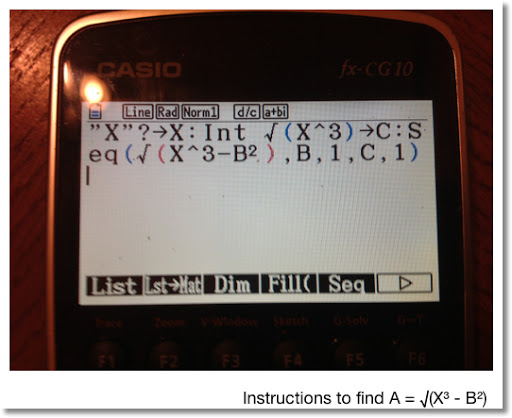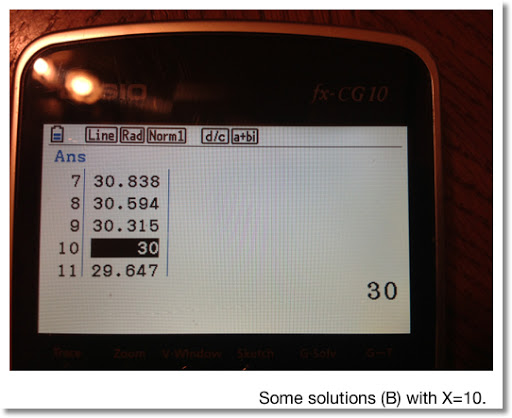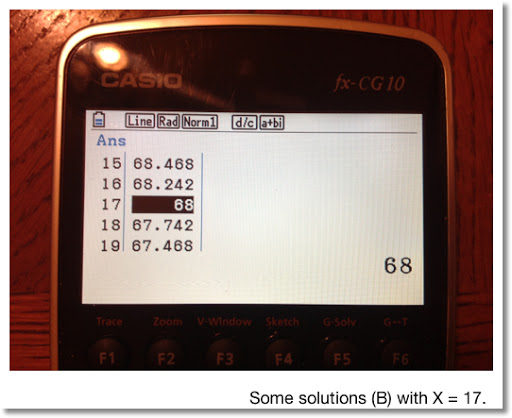Some solutions with X = 1 to 20... (A and B can be interchanged for (I))

(X=2, A=2, B=2)
8 = 2³ = 2² + 2² = 4 + 4

(X=5, A=2, B=11)
125 = 5³ = 2² + 11² = 4 + 121

(X=5, A=5, B=10)
125 = 5³ = 5² + 10² = 25 + 100

(X=8, A=16, B=16)
216 = 8³ = 16² + 16² = 256 + 256

(X=10, A=10, B=30)
1000 = 10³ = 10² + 30² = 100 + 300

(X=10, A=18, B=26)
1000 = 10³ = 18² + 36² = 324 + 676

(X=13, A=9, B=46)
2197 = 13³ = 9² + 46² = 81 + 2116

(X=13, A=26, B=39)
2197 = 13³ = 26² + 39² = 676 + 1521

(X=17, A=17, B=68)
4913 = 17³ = 17² + 68² = 289 + 4624

(X=17, A=47, B=52)
4913 = 17³ = 47² + 52² = 2209 + 2704

(X=18, A=54, B=54)
5832 = 18³ = 54² + 54² = 2916 + 2916

(X=20, A=16, B=88)
8000 = 20³ = 16² + 88² = 256 + 7744

(X=20, A=40, B=80)
8000 = 20³ = 40² + 80² = 1600 + 6400

(II) A² + B³ = X³

If A=0, then B=X, because B³ = X³. For B, X ∈ N.

If B=0, then A² = X³. Some solutions are:
A=1, X=1
A=8, X=4

Let A ≠ 0 and B ≠ 0. Solving for A yields A = √(X³ - B³). Here is the algorithm I used with the my Casio Prizm: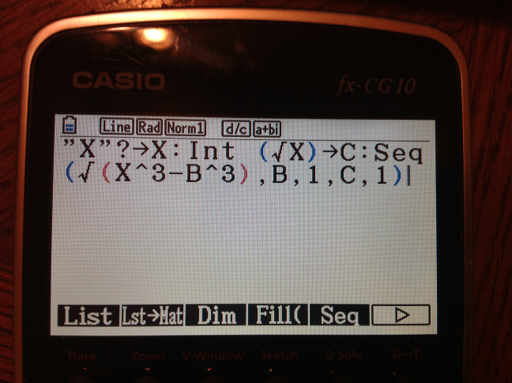Looking for integer solutions for X=1 to 20, yielded none. Apparently, solutions only exist when either A or B is allowed to be zero.

(III) A³ + B³ = X²

If A=0, then B ³ = X ². Likewise, if B=0, then A³ = X².

Without loss of generality, let A = 0. Some solutions are:

(A=0, B=4, X=8)
64 = 4³ = 8²

(A=0, B=6, X=16)
216 = 6³ = 16²

(A=0, B=16, X=64)
4096 = 16³ = 64²

Now assume, once again, that A ≠ 0 and B ≠ 0. Using the same strategies as (I) and (II), let's solve for A. Then A = ∛(X² - B³). Here is the algorithm I used with the Casio Prizm: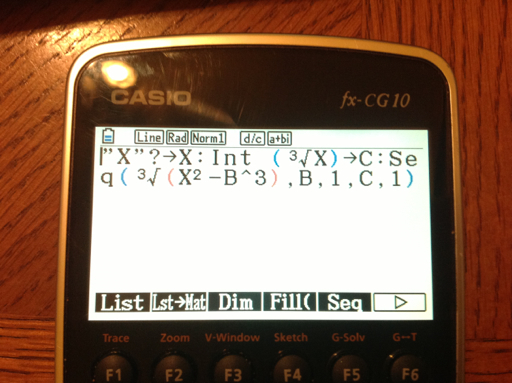The only solution that I found with X = 1 to 20 is:

(A=1, B=2, X=3). [A and B can be interchanged]
9 = 3² = 1³ + 2³ = 1 + 8

Stay safe and take care, Eddie. Thanks everyone.

This blog is property of Edward Shore. 2012

## Sunday, December 23, 2012

### More Equations Involving Natural Numbers

Greetings!

For the following let a, b, and n ∈ N. That is all variables are natural numbers (the counting numbers). Note that the set of natural numbers may or may not include 0. I will include 0 for this blog entry. This blog entry is all about trying to find solutions to equations - in an analytic manner.

a + b = a * b

If a = b, then:
a + a = a * a
2a = a^2

The only solution to this is a=2. (2*2 = 2^2 = 4)

Allowing for possibilities that a and b are different:
a + b = a * b
a + b - a * b = 0
a * (1 - b) = -b
a = -b/(1 - b) = b/(b - 1) = 1 + 1/(b - 1)

The only way that 1/(b - 1) ∈ N is when b = 2. Then a = 2.

The only solution to a + b = a * b is a = b = 2.

a^2 + b^2 = a * b

Let's say if a = b. Then:

a^2 + a^2 = a * a
2a^2 = a^2
a^2 = 0
a = 0

This implies that b=0. This works because 0^2 + 0^2 = 0 * 0 = 0.

What if we allow the possibility that a ≠ b?

a^2 + b^2 - a*b = 0
a^2 * (1 - b/a) + b^2 = 0

This lead me to believe that b is a multiple of a. Let b = a * n. Then:

a^2 * (1 - n) + a^2 * n^2 = 0

Assuming a ≠ 0,
(1 - n) + n^2 = 0

n = (1 ± i √3)/2.

Not good for searching for solutions such that a,b ∈ N.

In the general case:
a^2 + b^2 - a*b = 0
(1)(a^2) - (b)(a) + (b^2) = 0

Then:
a = (b ± √(b^2 - 4b^2))/2 = (b ± i b √3)/2 = b * (1 ± i √3)/2 which is not a natural number.

The only solution to a^2 + b^2 = a*b is a=b=0

a + b^2 = a * b

a + b^2 - a*b = 0
(1)(b^2) - (a)(b) + (a) = 0

b = (a ± √(a^2 - 4a))/2 = a/2 ± 1/2 * √(a^2 - 4a)

This implies that:
(1) a^2 - 4a must be a perfect square,
(2) a^2 - 4a must be even, and
(3) a must be even.

If a=2: √(2^2 - 4*2) = √(-4) = 2i. So a=2 is not a solution.

If a=4: √(4^2 - 4*4) = 0 which leads to b = 4/2 ± 0 = 2.
Then with a=4 and b=2:
4 + 2^2 = 4 * 2
8 = 8

A solution is found! Are there any more?

If a = 6, then a^2 - 4a = 12, not a perfect square.
If a = 8, then a^2 - 4a = 32, not a perfect square.
If a = 10, then a^2 - 4a = 60, not a perfect square.
If a = 12, then a^2 - 4a = 96, not a perfect square.
If a = 14, then a^2 - 4a = 140, not a perfect square.
If a = 16, then a^2 - 4a = 192, not a perfect square.

If a^2 - 4a is a prefect square, then a^2 - 4a = n^2. Then:
a^2 - 4a - n^2 = 0.
Then a = 2 ± √(4 + 2n^2). This is inconclusive.

One solution to a + b^2 = a * b is a=4 and b=2. There could be more.

a^2 + b^2 = 2 * a * b

Let's solve the equation in terms of b:
a^2 - 2 * a * b + b^2 = 0
(1)(b^2) - (2 * a)(b) + (a^2) = 0

And:
b = (2 * a ± √(4 * a^2 - 4 * a^2))/2 = (2 * a ± √0)/2 = a

This makes sense because when a=b, a^2 + a^2 = 2 * a * a = 2 * a^2.

Testing a few solutions:

a=3 and b=3:
3^2 + 3^2 = 18 and 2*3*3 = 18

a=6 and b=6:
6^2 + 6^2 = 72 and 2*6*6 = 72

The solution to a^2 + b^2 = 2*a*b is when a = b

Enjoy!

Eddie

This blog is property of Edward Shore. 2012

## Wednesday, December 19, 2012

### Equations without Whole Number Solutions - Fruitless Search?

Hi everyone! Hopefully you are fine today. Not long until 2013. And yes, I am 99.44% confident that the human race will still be on Earth be here come 12/22/2012.

x + x^2 = n^2

Let n,x ∈ N. N represents the natural numbers. Natural numbers are commonly referred to the whole numbers 1, 2, 3, etc. Some mathematicians include 0.

Are there any integer solutions to x + x^2 = n^2 with n,x ∈ N?

Our first instinct is most likely to go grab the nearest calculator or computer. Observe that:

(I). x + x^2 = n^2

(II). x^2 * (1/x + 1) = n^2

Taking the (principal) square root of both sides yields:

(III). x * √(1/x + 1) = n

In order for (III) to be true:
1. The quantity 1/x + 1 has to be an integer,
2. 1/x + 1 has to be a perfect square, and
3. x = 1/x + 1

The only natural number that allows condition 1 to be true is when x = 1.

Then 1/x + 1 = 2.

We know that 2 is not a perfect square (√2 ≈ 1.41421), so condition 2 fails. (II) does not fit because 1 * √2 = √2, leaving n = √2, not fitting the requirement that n,x ∈ N.

According to this analysis, there are no natural number solutions to x + x^2 = n^2.

x + x^2 + x^3 = n^q

Let n,x ∈ N. Suppose q = 2. Can we find solutions with these conditions?

x + x^2 + x^3 = n^2

x^2 * (1/x + 1 + x) = n^2

x * √(1/x + 1 + x) = n

Once again the only way 1/x + 1 + x is an integer is that when x = 1. However when x=1, 1/x + 1 + x = 3, and √3 ≈ 1.73205. And 1 * √3 = √3, n = √3, which is not a natural number.

There are no solutions (in the natural number set) for x + x^2 + x^3 = n^2.

Then x + x^2 + x^3 = n^3

x^3 * (1 + 1/x + 1/(x^2)) = n^3

x * ∛(1 + 1/x + 1/(x^2)) = n

Again, the only possible candidate is when x=1, but that leaves n = ∛3. No solutions in the natural number set.

Not all is "lost"...

Consider trying to find solutions to:

x + x^2 + x^3 + x^4 = n^2 where n,x ∈ N.

Then x * √(1/x + 1 + x + x^2) = n.

If x = 1, then √(1/x + 1 + x + x^2) = √4 = 2, and n = 1 * 2 = 2. Success! I believe x=1 and n=2 is the only solution to this equation with these conditions imposed.

Happy Holidays everyone and see you next time!

Eddie

This blog is property of Edward Shore. 2012

## Sunday, December 16, 2012

### Adding Numbers and their Reverses

Please take a look at the Fun With Num3ers blog by Benjamin Vitale (Twitter: @BenVitale). This is a fun blog working with number theory. It is also an inspiration for me to blog. Thanks, Ben!

Eddie

On to today's blog entry!

Two Digit Numbers

Let AB represent a two digit number, where A and B each represent a digit 0 through 9 (excluding 00). My original goal was to find a number AB such that AB + BA = n where n contains both digits A and B. I was unsuccessful.

However, I did see something curious:

11 + 11 = 22
12 + 21 = 33
13 + 31 = 44
14 + 41 = 55
15 + 51 = 66
16 + 61 = 77
17 + 71 = 88
18 + 81 = 99
19 + 91 = 110
10 + 01 = 10 + 1 = 11

All the sums for this group are multiples of 11.

If I go on...
21 + 12 = 33
22 + 22 = 44
23 + 32 = 55
24 + 42 = 66
25 + 52 = 77
....

Once again, multiples of 11. Can we show that this is true for all sums AB + BA?

Let a represent the digit A. Similarly, let b represent the digit B. Then:

AB = (10a + b) and BA = (10b + a)

Then the sum AB + BA = (10a + b) + (10b + a) = 11a + 11b = 11(a + b)

Three Digit Numbers

Let's consider the number ABC. Rotate ABC one digit and add. Repeat. We get the sum ABC + BCA + CAB = n.

Let a, b, and c represent the digits A, B, and C, respectively. Then ABC = 100a + 10b + c. We can construct two similar sums for BCA and CAB.

Then

ABC + BCA + CAB
= (100a + 10b + c) + (100b + 10c + a) + (100c + 10a + b)
= 111a + 111b + 111c
= 111(a + b + c)

The sum is ends up a multiple of 111, but not necessarily of 11. (111 is not a multiple of 11). Examples?

Example 1:
425 + 254 + 542 = 1221
1221/111 = 11
1221/11 = 111

Example 2:
963 + 639 + 396 = 1998
1998/111 = 18
1998/11 = 181 6/11
1998 is not a multiple of 11

Can we find such triples ABC, BCA, and CAB such that the sum is divisible by both 11 and 111? Hint: the least common multiple of 11 and 111 is 1,221. I have found 31 such triplets (including the trivial 0,0,0).

If you want to find the triples, have fun! I'll leave you with a few examples:

29 + 902 + 290 = 1221. (029, 902, 290)
137 + 713 + 371 = 1221. (137, 713, 371)
598 + 859 + 985 = 2442. (598, 859, 985)
254 + 425 + 542 = 1221. (254, 425, 542)

Take care and be safe!

Eddie

This blog is property of Edward Shore. 2012

## Saturday, December 15, 2012

### Trigonometric Functions with Random Number Arguments

Sine

Let R be a random number such that 0 ≤ R ≤ 1.

Then 0 ≤ R x ≤ x.

Since sin(0) = 0, we can simply conclude that 0 ≤ sin(R x) ≤ sin x, right?

However, we know that -1 ≤ sin x ≤ 1. Does this fact invalidate the claim that 0 ≤ sin(R x) ≤ sin x? Let's take a look at sin x vs sin(R x) graphically.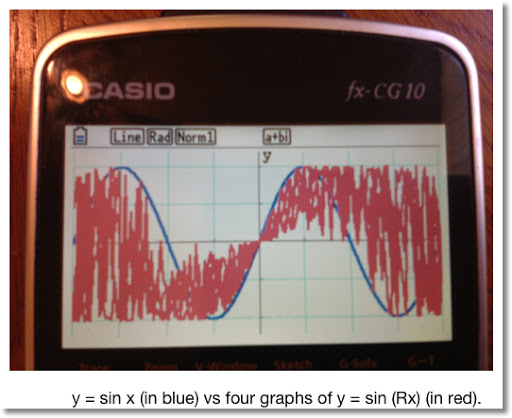From the graph, we can clearly see that 0 ≤ sin (Rx) ≤ sin x. Let's take a look at the region x ∈ [0, 2π].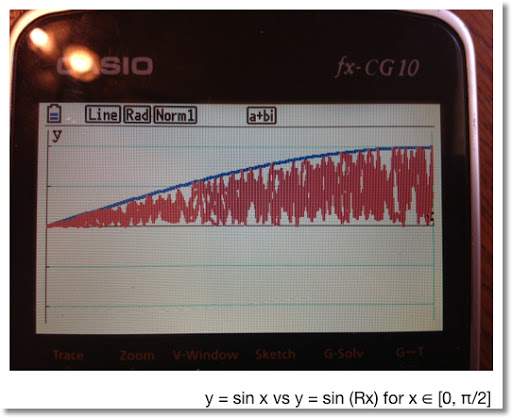In the region x ∈ [0, π/2], we can clearly see that 0 ≤ sin (Rx) ≤ sin x.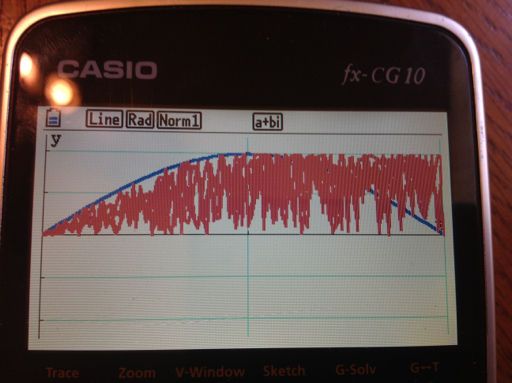As we explore the next region x ∈ [0, π], we still get positive values for sin (Rx). However, since R takes the value between 0 and 1, we get values 0 ≤ sin (Rx) ≤ 1.

Case: if R = 0.5 and x = π, sin(Rx) = 1.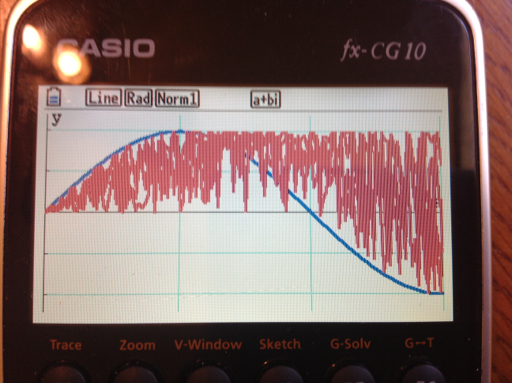When x ∈ [π/2, 3 π/2], the value of sin x declines below 0. However, since R is random, sin(Rx) takes the values sin x to 1.

Note that when x = 3π/2, sin(x) = -1.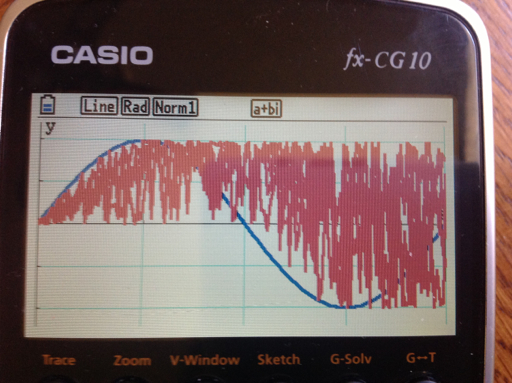At the final sub interval x ∈ [3π/2, 2 π], sin (Rx) takes on all values in the interval [-1, 1].

To summarize:

When 0 ≤ x ≤ π/2, 0 ≤ sin (Rx) ≤ sin x.
When π/2 ≤ x ≤ π, 0 ≤ sin (Rx) ≤ 1.
When π ≤ x ≤ 3π/2, sin x ≤ sin (Rx) ≤ 1.
When 3π/2 ≤ x ≤ 1, -1 ≤ sin (Rx) ≤ 1.

Interesting that we can't just say 0 ≤ sin (Rx) ≤ sin x for all x.

Cosine

We can make the similar argument that even though:

0 ≤ R ≤ 1, 0 ≤ R x ≤ x, and cos(0) = 1,

The statement 1 ≤ cos(Rx) ≤ cos x is not true for all x. Looking the graph cos x vs cos (Rx):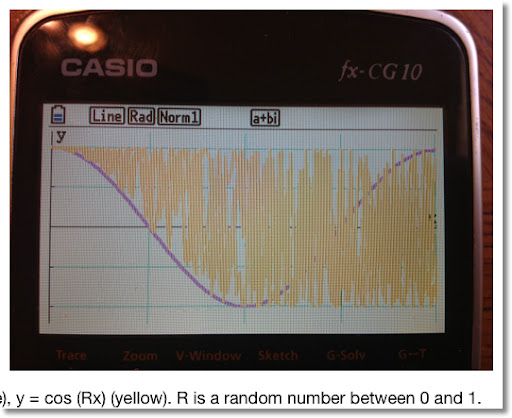Looking at the sub-intervals of [0, 2π]:

When 0 ≤ x ≤ π/2, cos x ≤ cos (Rx) ≤ 1.
When π/2 ≤ x ≤ π, π/2 ≤ cos (Rx) ≤ 1.
When π ≤ x ≤ 3π/2, -1 ≤ cos (Rx) ≤ 1.
When 3 π/2 ≤ x ≤ π, -1 ≤ cos (Rx) ≤ 1.

When random numbers are involved with functions, interesting things happen.

sin (Rx) cos (Rx) vs. 1/2 sin (2Rx)

Using the trig identity:

(sin x) * (cos x) = 1/2 * sin(2x)

Let R represent a random number function which returns a random number between 0 and 1. On a calculator, each time the random number function is called, a different random number is returned.

I will leave this as an open question: what happens at each interval [0, π/2], [π/2, π], [π, 3π/2], and [3π/2, 2 π]? I'll end this blog entry with a graphing comparison.

Note the range of sin(Rx) cos(Rx) is [-1, 1].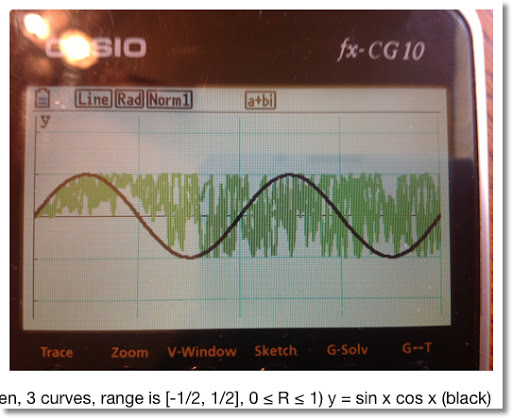Stay safe everyone! Eddie

This blog is property of Edward Shore. 2012

## Friday, December 14, 2012

### Random Numbers and Polynomials

Random Numbers and Polynomials

Let R be a random number such that 0 ≤ R ≤ 1. Then the inequality by x four times. Then:

0 ≤ R ≤ 1

0 ≤ R x ≤ x

0 ≤ R x^2 ≤ x^2

0 ≤ R x^3 ≤ x^3

0 ≤ R x^4 ≤ x^4

And for any integer n,

0 ≤ R x^n ≤ x^n

If we try to graph R x on a graphing calculator, we can imagine that the calculator generates a random R between 0 and 1 for each x. Graphing four y = R x on top of each other produces a result like this:Here are the graphs for y = R x^2, and y = R x^3, respectively.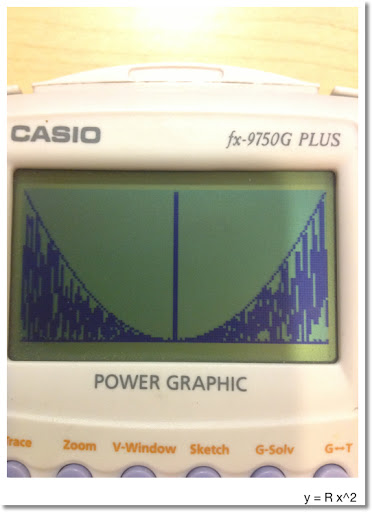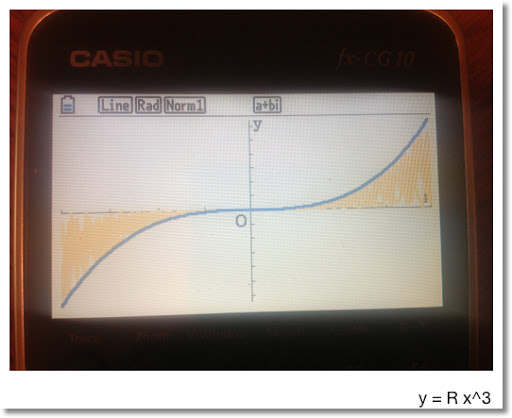Note that:

For odd n and x ≥ 0:

0 ≤ R x^n ≤ x^n

For odd n and x < 0:

0 ≥ R x^n ≥ x^n

For even n:

0 ≤ R x^n ≤ x^n

An Interesting Thing When Building Polynomials

Consider the function: y = Rx^2 + Rx + R

We can look at this two ways:

1. Treat R as a constant random number. Then y = R(x^2 + x + 1)

2. Treat each R as a separate random number, 0 ≤ R ≤ 1. In fact, when you put the function y = Rand# x^2 + Rand# x + Rand#, the calculator treats Rand# as a separate command.

Let's look at a comparison, where y = Rand# (x^2 + x + 1) is in red and y = Rand# x^2 + Rand# x + Rand# is in blue. Recall that Rand# is a random number function.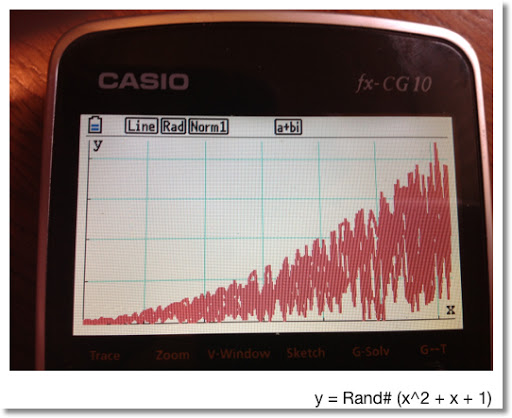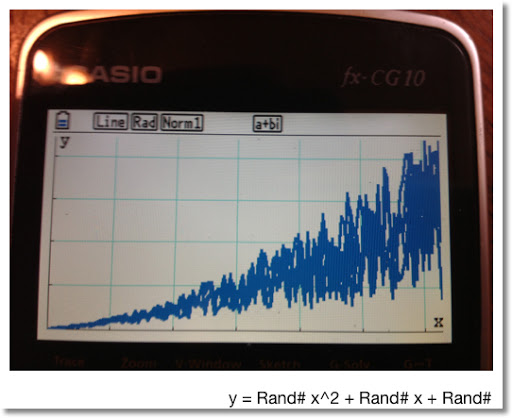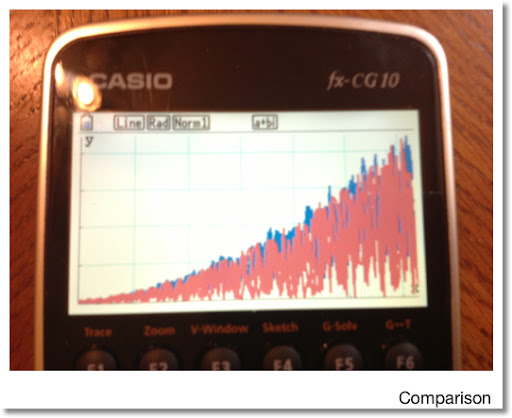That is it. Stay safe everyone, it is a crazy world! Eddie

This blog is property of Edward Shore. 2012

## Wednesday, December 12, 2012

### Happy 12/12/12 Day!

Celebrate the number 12 today! Have a great day everyone. Eddie

## Wednesday, December 5, 2012

### Numeric CAS - Part 12: Roots of a Complex Numbers

Complex Roots

Goal: Find all the complex roots of the complex number z.

This the solution to the equation:

x^n - z = 0

Where z = a + bi

Using de Moivre's Theorem:

Let r = abs(z) = √(x^2 + y^2) and
θ = arg(z) = arctan(y/x) where -π/2 ≤ θ ≤ π/2,

Then z^(1/n) = r^(1/n) * cos((2 k π + θ)/n) + i * r^(1/n) * sin((2 k π + θ)/n)
Where k = 0, 1, ... , n-1

Example:
Find the three roots of 1. ( ∛1 ) (n=3)

Roots: ≈ 1, -0.5 + 0.8660i, -0.5 - 0.8660i

Find the four roots of 2 + 7i. (n=4)

Roots: ≈ 1.5576 + 0.5216i, -0.5216 + 1.5576i, -1.5576 - 0.5216i, 0.5216 - 1.5576i

Casio Prizm:

CROOTS
Complex Roots
11/23/2012

(Prizm, fx-9860g, fx-9750gii - 92 bytes)
a+bi
"a+bi"? → Z
"ROOT"? → N
For 0 → K To N-1
N x√ (Abs Z) × e^( i (Arg Z + 2Kπ) ÷ N) ◢
Next

Alternative (done on CFX-9850G, older Casios - 94 bytes)
"A+Bi"? → Z
"ROOT"? → N
For 0 → K To N-1
N x√ (Abs Z) × ( cos ((Arg Z + 2Kπ) ÷ N) + i sin ((Arg Z + 2Kπ) ÷ N)) ◢
Next

TI-84+:

CROOTS
Complex Roots (TI-83+/TI-84+)
11/23/2012
76 Bytes

a+bi
Input "a+bi:", Z
Input "ROOT:", N
For(K,0,N-1)
Pause N x√ (abs(Z)) * e^(i(angle(Z)+2Kπ )/N)
End

HP 39gii:

PROGRAM CROOTS
All the N complex roots of Z
11/23/2012

Input: CROOTS(Z,N)

EXPORT CROOTS(Z,N)
BEGIN
LOCAL L3,K;
0 → HAngle; // set calculator to Radians mode
{ } → L3;
FOR K FROM 0 TO N-1 DO
CONCAT(L3, {N NTHROOT (ABS(Z)) * e^( i ( ARG(Z) + 2 * K * π )/N) → L3;
END;
RETURN L3;
END;

This concludes the Numeric CAS series... For now.

Until next time, Eddie

This blog is property of Edward Shore. 2012

### Numeric CAS - Part 11: Eigenvalues of 3 x 3 Matrices

Eigenvalues of 3 x 3 Matrices

Many graphing calculators that do not have CAS (computer algebraic systems) do not have eigenvalues and eigenvector functions.

The programs for the Casio Prizm and TI-84+ gives eigenvalues of 3 x 3 matrices.

For the HP 39gii has the functions EIGENVAL for eigenvalues.

Example:
A = [[2, -2, 3][-1, 0, 1][6, -3, 3]]

Eigenvalues:
≈ 0.2465, -1.8447, 6.5982

Casio Prizm:

EIGEN3
Eigenvalues of a 3 × 3 Matrix
Circa 2011 - 244 bytes

a+bi
"3 × 3 Matrix"? → Mat A
Mat A[1,1] + Mat A[2,2] + Mat A[3,3] → T
(Mat A)² → Mat B
Mat B[1,1] + Mat B[2,2] + Mat B[3,3] → U
Solve(-X^3 + X^2 × T + X × 1/2 × (U-T^2) + Det Mat A,0)→ R ◢
-R^2 + R × T - T^2 ÷ 2 + U ÷ 2 → A
-R + T → B
1/2 × (B - √(4A + B^2)) → E ◢
1/2 × (B + √(4A + B^2)) → F ◢
"STORED IN R, E, F"

TI-84+:

EIGEN3
Eigenvalues of a 3 × 3 matrix - 193 bytes
12/3/12

a+bi
Input "3 X 3 MATRIX:", [A]
[A](1,1) + [A](2,2) + [A](3,3) → T
[A]² → [B]
[B](1,1) + [B](2,2) + [B](3,3) → U
solve(-X³ + X² T + .5X(U-T²) + det([A]), X, 0) → R
Pause R
-R² + RT - T²/2 + U/2 → A
-R + T → B
.5(B - √(4A+B²)) → E
.5(B + √(4A+B²)) → F
Pause E
Pause F

This blog is property of Edward Shore. 2012

### Numeric CAS Part 10 - Eigenvalues of 2 x 2 Matrices

Eigenvalues of 2 x 2 Matrices

Many graphing calculators that do not have CAS (computer algebraic systems) do not have eigenvalues and eigenvector functions.

The program for the Casio Prizm gives eigenvalues. The TI-84+ program gives both eigenvalues and eigenvectors.

For the HP 39gii has the functions EIGENVAL and EIGENVV for eigenvalues and eigenvectors, respectively.

Example:
A = [[2, 7][-3, 9]]

Eigenvalues: ≈ 5.5 ± 2.9580i

Casio Prizm:

Eigenvalues of a 2 × 2 Matrix
EIGEN2 - 136 Bytes

a+bi
"2 × 2 MATRIX:"? → Mat A
Mat A[1,1] + Mat A[2,2] → T
Det Mat A→ D
(T+√(T^2-4D))÷2 → R ◢
(T-√(T^2-4D))÷2 → S ◢
"λS STORED IN R,S"

TI-84+:

EIGEN2
Eigenvalues of 2 × 2 matrices - Approximately 280 bytes
Bonus! A pair of eigenvectors are given
5/15/2011

a+bi
Input "2 X 2 MATRIX:", [J]
[J](1,1) + [J](2,2) → T
det([J]) → D
(T + √(T^2 - 4D))/2 → U
(T - √(T^2 - 4D))/2 → V
Disp "EIGENVAL. U"
Pause U
(U - [J](1,1)) / [J](1,2) → A
√(1 + A^2)⁻¹ * [[A]] → [H]
Disp "EIGENVEC. [H]"
Pause [H]
(V - [J](1,1)) / [J](1,2) → B
√(1 + B^2)⁻¹ * [[B]] → [I]
Disp "EIGENVAL. V"
Pause V
Disp "EIGENVECT. [I]"
Pause [I]

This blog is property of Edward Shore. 2012

### Numeric CAS - Part 9: Indefinite Integrals of Polynomial

Indefinite Integral of a Polynomial

Goal: Determine the coefficients of the indefinite integral of the polynomial p(X) where

p(X) = a_n * x^n + a_(n-1) * x^(n-1) + ... + a1 * x + a0

and

∫ p(X) dX = a_n * x^(n+1) ÷ (n+1) + a_(n-1) * x^n ÷ n + ... + a0 * x + c

Where c is a constant. This constant is determined by the initial condition (x_0, p_0). The programs assume that c = 0, which is common in computer algebraic systems.

Where a_n, a_(n-1), ... , a1, a0 are stored in lists. The coefficients are listed in order of descending powers of x. Use zeros as placeholders.

Example:

∫ -2x^2 + 4x - 6 dx = -2/3 * x^3 + 2x^2 - 6x
Input List: {-2, 4, -6}
Output List: {-2/3, 2, -6, 0} or
{-0.666666667, 2, -6, 0}

∫ x^4 + 3x^2 - x dx = 1/5 * x^5 + x^3 - 1/2 * x^2
Input List: {1, 0, 3, -1, 0}
Output List: {1/5, 0, 1, -1/2, 0, 0} or
{0.2, 0, 1, -0.5, 0, 0}

For all three programs, the input is List 1, the output is List 2.

Casio Prizm:

POLYINT
Indefinite Polynomial Integral - 140 bytes

"∫ (P(X)) dX"
"{AnX^n,...,A0}"
"LIST="?→List 1
List 1 → List 2
For 1→K To Dim List 1
List 2[K] ÷ (Dim List 2 + 1 - K) → List 2[K]
Next
Augment(List 2,{0}) → List 2
List 2

TI-84+:

POLYINT
Polynomial Indefinite Integral - 117 bytes

: Disp "fnInt(P(X)) DX", "{AnX^n,...,A0}"
: Input "LIST:", L1
: L1 → L2
: For(K,1,dim(L2))
: L2(K)/(dim(L2)-K+1) → L2(K)
: End
: augment(L2,{0}) → L2
: Pause L2

HP 39gii:

Polynomial Integral
POLYINT()
Same input as above

EXPORT POLYINT()
BEGIN
LOCAL K,S;
EDITLIST(L1);
SIZE(L1)→S;
{ } → L2;
FOR K FROM 1 TO S DO
CONCAT(L2, {(S-K+1)⁻¹ * L1(K)}) → L2;
END;
CONCAT(L2,{0})→ L2;
RETURN L2;
END;

This blog is property of Edward Shore. 2012

### Numeric CAS - Part 8: Polynomial Derivative

Derivative of a Polynomial

Goal: Determine the coefficients of the derivative of the polynomial p(X) where

p(X) = a_n * x^n + a_(n-1) * x^(n-1) + ... + a1 * x + a0

and

d/dX p(X) = a_n * n * x^(n-1) + a_(n-1) * (n-1) * x^(n-2) + ... + a1

Where a_n, a_(n-1), ... , a1, a0 are stored in lists. The coefficients are listed in order of descending powers of x. Use zeros as placeholders.

Example:

d/dx -2x^2 + 4x - 6 = -4x + 4
Input List: {-2, 4, -6}
Output List: {-4, 4}

d/dx x^4 + 3x^2 - x = 4x^3 + 6x - 1
Input List: {1, 0, 3, -1, 0}
Output List: {4, 0, 6, -1}

For all three programs, the input is List 1, the output is List 2.

Casio Prizm:

POLYDX
Polynomial Derivative - 132 bytes

"d/dX P(X)"
"{AnX^n,...,A0}"
"LIST:"? → List 1
Dim List 1-1 → Dim List 2
For 1→K To Dim List 2
(Dim List 1-K) × List 1[K] → List 2[K]
Next
List 2

TI-84+:

POLYDX
Polynomial Derivative - 118 bytes

: Disp "D/DX P(X)", "{AnX^n,...,A0}"
: Input "LIST:",L1
: L1->L2
: dim(L2)-1->dim(L2)
: For(K,1,dim(L2))
: (dim(L2)-(K-1))*L2(K)->L2(K)
: End
: Pause L2

HP 39gii:

Polynomial Derivative
11/23/2012 - edited 12/2/2012

POLYDX()

EXPORT POLYDX()
BEGIN
LOCAL K,S;
EDITLIST(L1);
SIZE(L1)→S;
{ } → L2;
FOR K FROM 1 TO S-1 DO
CONCAT(L2, {(S-K)*L1(K)}) → L2;
END;
RETURN L2;
END;

This blog is property of Edward Shore. 2012

## Monday, December 3, 2012

### Numeric CAS - Part 7: Quadratic Equation

Ax² + Bx + C = 0

Where x = (-B ± √(B² - 4AC)) / (2A)

This is just the popular quadratic formula, except this set of programs allow for complex A, B, and C. (Unlike the Solver applications).

Example:
A = 2 - i
B = -6
C = 14i

x1 ≈ -0.9873 + 1.551i
x2 ≈ 3.3873 - 0.3509i

Casio Prizm:

Quadratic Equation - Complex Coefficients Allowed
11/23/2012 - 124 bytes

a+bi
"AX²+BX+C=0"
"A"? → A
"B?"→ B
"C?"→ C
√(B²-4AC) → X
(-B - X) ÷ (2A)→ R
(-B + X) ÷ (2A) → S
"X=R Or X=S"
R ◢
S

TI-84+:

Quadratic Formula - Allows for Complex Coefficients
Ax^2 + Bx + C = 0

a+bi
Disp "AX²+BX+C"
Prompt A,B,C
√(B²-4AC) → X
(-B - X)/(2A) → R
(-B + X)/(2A) → S
Disp "X=R or X=S"
Pause R
Pause S

HP 39gii:

Use the POLYROOT command. POLYROOT([A,B,C])

The vector can represent any polynomial.

This blog is property of Edward Shore. 2012

### Numeric CAS Part 6: Euclid Algorithm to find GCD (Greatest Common Divisor)

Euclid Algorithm

Goal: To find the greatest common divisor of two integers U and V.

The Algorithm in Brief:

Let U ≥ V. Then U = A * V + R where A is the quotient and R is the remainder. If R≠0, then V becomes the new U, and R becomes the new V. The process repeats until you R=0. When that happens, V_last = GCD(U,V).

The program allows for you two enter integers in any order. Each program uses the home screen to display the steps, but the HP 39gii displays all the steps on one screen.

Example: gcd(166, 78)

166 = 2 * 78 + 10
78 = 7 * 10 + 8
10 = 1 * 8 + 2
8 = 4 * 2 + 0
GCD = 2

gcd(169,39)

169 = 4 * 39 + 13
39 = 3 * 13 + 0
GCD = 13

Casio Prizm:

EUCLID
GCD Program - 12/3/12
212 bytes

"X"?→ X
"Y"?→ Y
If X ≥ Y
Then X → M
Y → N
Else X → N
Y → M
IfEnd
Lbl 1
Int(M ÷ N) → A
M - A × N → R
ClrText
Locate 1,2,M
Locate 1,3,"="
Locate 2,3,A
Locate 13,3,"×"
Locate 14,3,N
Locate 1,4,"+"
Locate 2,4,R ◢
If R=0
Then Goto 2
Else N → M
R → N
Goto 1
IfEnd
Lbl 2
Locate 1,6,"GCD="
Locate 6,6,N

TI-84+:

GCD by Euclidian Algorithm
11/25/2012 - 160 bytes

Program EUCLID

Prompt X,Y
min(X,Y) → N
max(X,Y) → M
Lbl 1
iPart(M/N) → A
M - A * N → R
ClrHome
Output(2,1,M)
Output(3,1,"=")
Output(3,2,A)
Output(3,8,"*")
Output(3,9,N)
Output(4,1,"+")
Output(4,2,R)
Pause
If R=0
Then
Goto 2
Else
N → M
R → N
Goto 1
End
Lbl 2
ClrHome
Disp "GCD=", N

HP 39gii:

Program EUCLID
Finding the GCD by the Euclid algorithm
10/16/2012

Input: EUCLID(X,Y)

EXPORT EUCLID(X,Y)
BEGIN
LOCAL M,N,R,A;
MAX(X,Y)→ N;
MIN(X,Y)→M;
PRINT();
REPEAT
INT(N/M)→ A;
N - A*M→ R;
PRINT(A + "*" + M + "+" + R);
WAIT(1);
M→ N;
R→ M;
UNTIL R==0;
RETURN N;
END;

This blog is property of Edward Shore. 2012

## Sunday, December 2, 2012

### Numeric CAS - Part 5: Polynomial Evaluation

Polynomial Evaluation

Goal: Evaluation of the polynomial p(x) at x = a. This gives an alternative to typing out the polynomial itself.

Enter the coefficients of the polynomial as a list, in descending order from highest power to constant. Use zeros as place-holders.

Example:
Let p(x) = 2x^4 + 2x^2 - 4x + 1 at x = -1.5

List Input: {2, 0, 2, -4, 1}
Enter -1.5 when the program asks for X.

Result: p(-1.5) = 21.625

Casio Prizm:

POLYEVAL
Poly Evaluation
11/23/2012
(140 bytes)

Example: p(x) = x^3 + 2x^2 + 5x + 9, p(-2) = -1

Program:
"{AnX^n,...,A0}"
"P(X)"? → List 1
"X"? → X
X = 0 ⇒ Goto 1
0 → S
For 1 → K To Dim List 1
S + List 1[K] × X^(Dim List 1 - K) → S
Next
S
Stop
Lbl 1
List 1[Dim List 1]→ S

Sum is stored in S, 0^0 is not allowed.

TI-84+:

POLYEVAL
Polynomial Evaluation
11/23/2012 - 124 bytes

Example: p(x) = 1.425x^3 - 2.89x^2 + 0.23x + 4.4546
p(1.002) = 3.217055551

For the character n: VARS, 5, 1

Disp "{AnX^n,...,A0}"
Input "P(X):", L1
Input "X:", X
If X=0
Goto 1
0→ S
For(K,1,dim(L1))
S + L1(K) * X^(dim(L1) - K) → S
End
Pause S
Stop
Lbl 1
L1(dim(L1)) → S
Pause S

HP 39gii:

There is no program. Instead, use the POLYEVAL command.

POLYEVAL(vector, value)

The vector contains the coefficients of the polynomial.

This blog is property of Edward Shore. 2012

### Numeric CAS - Part 4: Polynomial Multiplication

Polynomial Multiplication

Goal: Multiply two polynomials: p1(x) * p2(x) = p3(x)

Inputs: Coefficients of p1(x) and p2(x) as List 1 and List 2, respectively

Output: Coefficients of p3(x) as List 3.

The lists are in order from highest order to constant. Zeros are used as place holders.

Example: (x^3 + 2x - 1) * (-2x^2 + x - 4) = -2x^5 + x^4 - 8x^3 + 4x^2 - 9x + 4

Input:
List 1: {1, 0, 2, -1}
List 2: {-2, 1, -4}

Output:
List 3: {-2, 1, -8, 4, -9, 4}

Casio Prizm:

POLYMULT
Multiplication of Two Polynomials
11/21/2012 - 176 bytes

"{AnX^n,...,A0}"
"P1(X)"? → List 1
"P2(X)"? → List 2
Dim List 1 + Dim List 2 - 1 → Dim List 3
For 1 → K To Dim List 1
List 1[K] × List 2 → List 4
For 1 → J To Dim List 4
List 3[J + K - 1] + List 4[J] → List 3[J + K - 1]
Next
Next
List 3

Example:
(x^2 + 3x - 1)(x^2 - 4) = x^4 + 3x^3 - 5x^2 - 12x + 4
List 1: {1,3,-1}
List 2: {1,0,-4}
Result List 3: {1,3,-5,-12,4}

TI-84+:

POLYMULT
Multiplication of two Polynomials
{AnX^n,...,A0}
11/21/2012 - 154 bytes

To get the lower case "n" character: VARS, 5, 1

Disp "{AnX^n,...,A0}"
Input "P1(X)=", L1
Input "P2(X)=", L2
dim(L1) + dim(L2) - 1 → dim(L3)
Fill(0,L3)
For(K, 1, dim(L1))
L1(K) * L2 → L4
For(J, 1, dim(L4))
L3(J + K - 1) + L4(J) → L3(J + K - 1)
End
End
Pause L3

HP 39gii:

Polynomial Multiplication (FULL)
12/2/2012

EXPORT POLYMULT()
BEGIN
LOCAL S,K,J;
EDITLIST(L1);
EDITLIST(L2);
SIZE(L1)+SIZE(L2)-1 → S;
MAKELIST(0,X,1,S,1) → L3;
FOR K FROM 1 TO SIZE(L1) DO
L1(K)*L2 → L4;
FOR J FROM 1 TO SIZE(L4) DO
L3(J+K-1) + L4(J) → L3(J+K-1);
END;
END;
RETURN L3;
END;

This blog is property of Edward Shore. 2012

### Numeric CAS - Part 3: Synthetic Division

Synthetic Division

Goal: Divide the polynomial p(x) = a_n x^n + a_(n-1) x^(n-1) + ... + a_0 by (x - r).

p(x) ÷ (x - r) = q_n x^n + q_(n-1) x^(n-1) + ... + q_0 with remainder E

Input: A list of coefficients {a_n, a_(n-1), a_(n-2), ... , a_0} (List 1)

Output: Resulting coefficients {q_n, q_(n-1), ... , q_0, E} (List 2)

Example: (2x^3 + x - 3) ÷ (x - 3) = 2x^2 + 6x + 19 + 54 ÷ (x - 3)

List 1: {2, 0, 1, -3}, R = 3
List 2: {2, 6, 19, 54}

Casio Prizm

POLYSYN
Synthetic Division - 160 bytes

"P(X) ÷ (X-R)"
"{AnX^n,...,A0}"
"LIST:"?→List 1
"R"?→R
List 1 → List 2
For 1→K To Dim List 1 - 1
R × List 2[K] + List 1[K+1] → List 2[K+1]
Next
"LAST TERM = REMAINDER" ◢
List 2

TI-84+

POLYSYN
Synthetic Division - 143 bytes

: Disp "P(X)/(X-R)"
: Disp "{AnX^n,...,A0}"
: Input "LIST:", L1
: Input "R:", R
: L1->L2
: For(K,1,dim(L1)-1)
: R*L2(K)+L1(K+1)->L2(K+1)
: End
: Disp "LAST TERM=", "REMAINDER"
: Pause L2

HP 39gii

POLYSYN
Synthetic Division
11/23/2012

L1 and A are prompted. The resulting is a list of coefficients, with the last term the remainder

Example: (21x^2 + 42x + 144)/(x - 12) = 21x + 294 + 3672/(x-12)
Output List: {21, 294, 3672}

EXPORT POLYSYN()
BEGIN
LOCAL K,S,T;
EDITLIST(L1);
INPUT(R,"P(X)/(X-R)");
L1 → L2;
SIZE(L1)→ S;
S-1 → T;
FOR K FROM 1 TO T DO
R * L2(K) + L1(K+1) → L2(K+1);
END;
MSGBOX("LAST TERM=REMAINDER");
RETURN L2;
END;

This blog is property of Edward Shore. 2012

## Saturday, December 1, 2012

### Numeric CAS Part 2: Binomial Expansion

Binomial Expansion

Goal: To find the coefficients of when (ax + by)^n is expanded. The variables a and b are numeric.

(ax + by)^n = Σ (n nCr k *(ax)^(n-k) * (by)^k for k = 0 to n).

Example: (3x - 2y)^3 = 27 x^3 - 54 x^2 y + 36 x y^2 - 8 y^3

For the Casio Prizm and TI-84+, each coefficient is given in order. They are also stored in List 6. For the HP 39gii, a string is built representing the expanded binomial.

The list in the above example is {27, -54, 36, -8}Casio Prizm:

POLYBINE
Binary Expansion
216 bytes

Lbl 6
"(AX+BY)^N"
"A"?→ A
"B"?→ B
"N"?→ N
If N≤0 Or Frac N≠0
Then
"N NOT AN INTEGER > 0" ◢
Goto 6
IfEnd
For 0 → K To N
ClrText
N nCr K × A ^ (N - K) × B ^ K → C
Locate 1,2,C
Locate 1,3,"×X^"
Locate 4,3,N-K
Locate 7,3,"×Y^"
Locate 10,3,K ◢
C→ List 1[K+1]
Next

Thanks to Ryan Maziarz for pointing out an extra quotation mark I had on line 3  (originally "A"?"A).  2/6/2013

Note:  The program will show the coefficients of the expansion one at a time.  You can view the entire list of the coefficients in List 1.

TI-84+:

POLYBINE
Binomial Expansion
177 bytes

: Lbl 1
: Disp "(AX+BY)^N"
: Prompt A,B,N
: If N≤0 or fPart(N)≠0
: Then
: Disp "NEED INTEGER>0"
: Pause
: Goto 1
: End
: N+1->dim(L6)
: For(K,0,N)
: N nCr K*A^(N-K)*B^K->C
: ClrHome
: Output(2,1,C)
: Output(3,1,"*X^")
: Output(3,4,N-K)
: Output(3,7,"*Y^")
: Output(3,10,K)
: Pause
: C->L6(K+1)
: End

Note:  The program will show the coefficients of the expansion one at a time.  You can view the entire list of the coefficients in L6.

HP 39gii:

POLYBINE
11/23/2012
Polynomial Binomial Expansion
Expand (ax + by)^n

The results are returned in a string. Note, if N is not a positive integer, it is converted into one.

Input: POLYBINE(A,B,C)

EXPORT POLYBINE(A,B,C)
BEGIN
LOCAL S1,S2,K;
ABS(INT(N)) → N;
"" → S1;
FOR K FROM 0 TO N DO
string(COMB(N,K)*A^(N-K)*B^K) → S2;
S1 + "+" + S2 + "*X^" + string(N-K) + "*Y^" + string(K) → S1;
END;
dim(S1) → K;
right(S1,K-1) → S1;
RETURN S1;
END;

This blog is property of Edward Shore. 2012

### Numeric CAS Part 1: Simplifying Square Roots

Simplifying Square Roots

Goal: Simplify square roots of integers. For example, √180 = 6 √5, √80 = 4 √5

General Algorithm: Start with integer N and C=1. Starting with k=2, divide N by k². If N divides k² evenly, N is adjusted, C is multiplied by k, and the division is test is repeated. If N does not not divide k², k increases by 1 and the division test repeats. The division tests repeat until k² > N.

The program for the Casio Prizm, TI-84+, and HP 39gii are presented below:

Casio Prizm:

SQFACTOR
11/19/2012
Simplifies √N where N is an integer (i.e. √180 = 6 √5, √364 = 2 √91)
156 bytes

"√N="? → N
N → M
1 → C
2 → K
Do
Lbl 1
If Frac(N ÷ K²)=0
Then
N ÷ K² → N
C × K → C
Goto 1
IfEnd
K + 1 → K
LpWhile K²ClrText
Locate 1,1,"√"
Locate 2,1,M
Locate 1,3,C
Locate 10,3,"×√"
Locate 12,3,N

TI-84+:

SQFACTOR
Square Root Simplification
(I.E. √180 = 6 √5 , √364 = 2 √91)
11/19/2012
133 bytes

Input "√(", N
N → M
1 → C
2 → K
Lbl 0
If fPart(N/K²)=0
Goto 1
1 + K → K
If K² < N
Goto 0
ClrHome
Output(1,1,"√(")
Output(1,3,M)
Output(3,1,C)
Output(3,7,"√(")
Output(3,9,N)
Stop
Lbl 1
N/K² → N
C*K → C
Goto 0

HP 39gii:

SQFACTOR
11/23/2012
Simplifies √N where N is an integer (i.e. √180 = 6 √5, √364 = 2 √91)

Input: SQFACTOR(N)

EXPORT SQFACTOR(N)
BEGIN
LOCAL C,K;
1 → C;
2 → K;
WHILE K² < N DO
WHILE FRAC(N/K²) == 0 DO
N/K² → N;
C*K→ C;
END;
K+1→K;
END;
RETURN string(C)+"√"+string(N);
END;

This blog is property of Edward Shore. 2012

### Numeric CAS Project - an Introduction

It's coming! The Numeric CAS project is a set of programs designed to give common graphing calculators a set of some common computer algebraic system functions and advanced graphing calculators.

The calculators that I will be working with in this series are:

* Texas Instruments TI-84 Plus
* Casio Prizm fx-CG 10 (should work on most Casio graphing calculators)
* Hewlett Packard 39gii

Everything is this series is done by using the calculator's native languages. No hacking is required. The programs use the numeric features of the calculator.

Often, lists will be used to represent coefficients of polynomials. The power of coefficients are in descending order. For example, to represent the polynomial x^3 + 2x - 1, the list would be {1, 0, 2, -1}. Zeroes are necessary in these lists.

There will be twelve parts in the Numerical CAS Project:

1. Simplifying Square Roots of Integers
2. Expanding Binomials
3. Synthetic Division
4. General Multiplication of Polynomials
5. Polynomial Evaluation
6. Euclid Algorithm to find GCD
8. Derivative of a Polynomial
9. Indefinite Integral of a Polynomial
10. Eigenvalues of a 2 x 2 Matrix
11. Eigenvalues of a 3 x 3 Matrix
12. Complex Roots of a Complex Number

I aim to post all twelve parts throughout December 2012. What is awesome about programming a calculator is that you extend its functionality beyond the already extensive list of given functions, and you don't have to be an expert in assembly or complex language.

Eddie

This blog is property of Edward Shore. 2012

## Sunday, November 25, 2012

### Two more calculators added to the collection.

I love swap meets and pawn shops. Eddie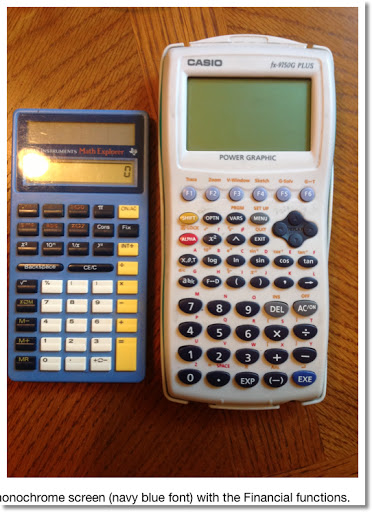### f(x) = x * random ^ random

Earlier this month, I received a request from Jason Foose. These are six graphs of y = x * rand^rand: two with the TI-84+, two with the Casio Prizm (fx-CG 10, and two with the HP 39gii.

On the Prizm, I had to use the catalog to find the random number function (labeled Ran#).

Re-editing the function will cause the function to be redrawn.

Enjoy!

Eddie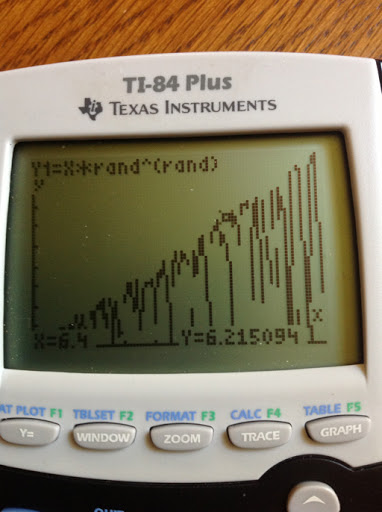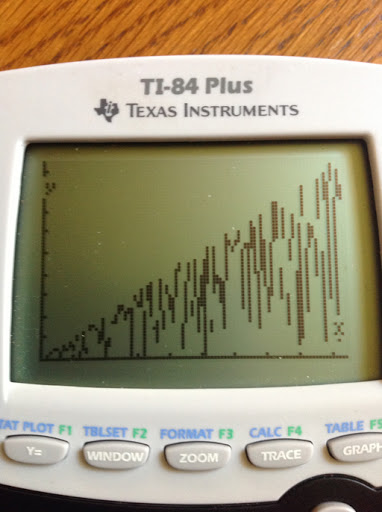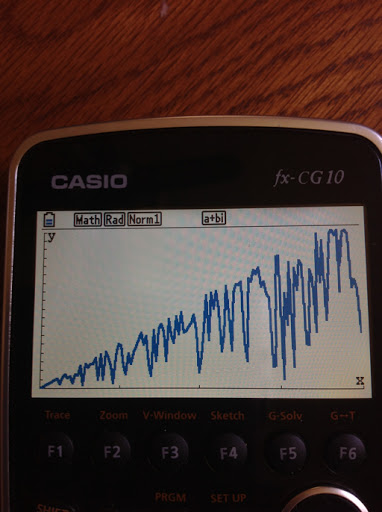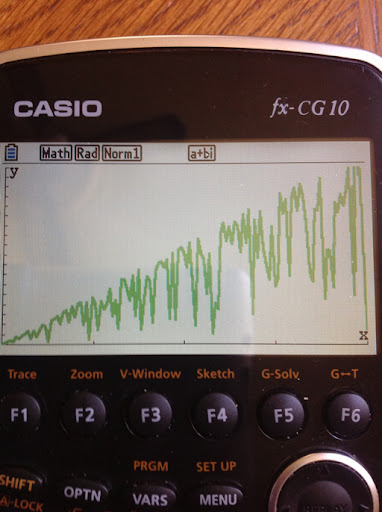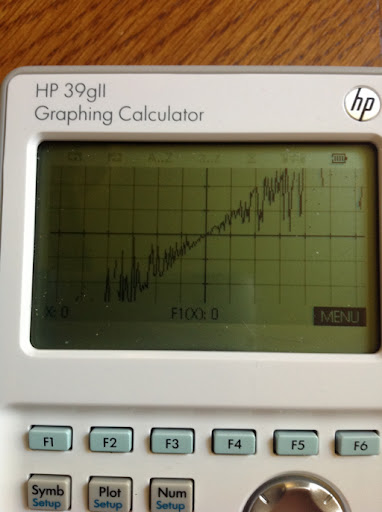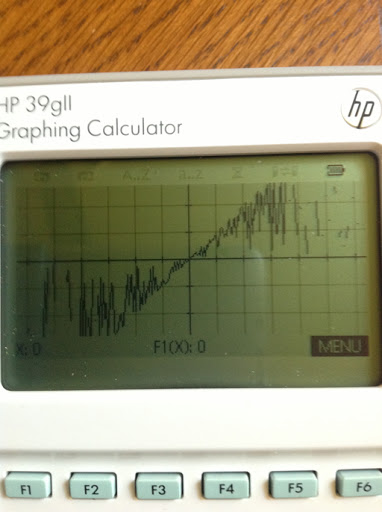### Matrix Functions on the fx-991ES (fx-115ES in the US) vs TI-36X Pro

Matrix Function Comparison (fx-991ES/fx-115ES vs TI 36X Pro)

Today's blog entry comes from a question by An Artist:

An Artist has left a new comment on your post "TI-36X Pro Review":

Hi Eddie,

In India, the TI 36x pro is available at the same price as the Casio 991ES.
I have ordered the TI.

Is this a good decision?
I've heard of a memory overload bug of the TI which gives wrong answers. Can you please tell me how to get around the bug?

My main focus is matrix and someone told me that this one has more matrix functions for matrices. Can you please confirm that?

First of all thank you for the compliment An Artist, very much appreciated.

Regarding the overload bug on the TI-36X Pro, honestly this is the first time I read of it. According to one of the reviewers on the flipkart.com page, the reviewer reports an overload bug occurs when too many equations are used or too much memory is used. I have not found any additional information about this. Personally I have not encounter this problem.

Now the matrix commands. I am going to list the available matrix functions for each calculator. First up, Casio, then Texas Instruments.

Keep in mind both calculators allow only for real-numbered elements.

Casio fx-991ES (aka fx-115ES in the United States)

You will need to enter a specific mode for Matrices (MODE 6)

3 matrices can be stored, up to size 3 x 3 matrices. A separate "Ans" matrix is also available.

Matrix Functions:
Arithmetic
Ability to resize matrices
Determinant (dim)
Transpose (Trn)
Inverse (with the x⁻¹ button)
Square of a square matrix (with the x² button)
Cube of a square matrix (with the x³ function)
Absolute Value of each element (Abs)

Matrices are automatically cleared when modes are switched.

Note: The updated fx-115ES PLUS adds the ref and rref functions.

Texas Instruments TI-36X Pro

There is no specific mode required, you can work with matrices directly from the Home screen.

3 matrices can be stored, up to size 3 x 3 matrices. A separate "Ans" matrix is also available. The TI-36X offers identity matrices [I2] and [I3] of size 2 x 2 and 3 x 3, respectively.

Matrix Functions:
Arithmetic
Ability to resize matrices (through the edit screen)
Determinant (dim)
Transpose (Trn)
Inverse
Square of a square matrix (with the x² button)
Powers of a square matrix ([A]^n where n is a positive integer, 0, or -1)
Absolute Value of each element (abs)
Integer Part of each element (iPart)
Fractional Part of each element (fPart))
Rounding of each element (round)
Reduced Echelon Form (ref)
Row Reduced Echelon Form (rref)

Matrices are retained in memory on the TI-36X Pro.

An Artist, to answer your question between the fx-991ES and the TI-36X Pro, I can confirm that the TI-36X Pro has more functions for matrices. Hope this helps,

Eddie

## Friday, November 23, 2012

### My next blog series?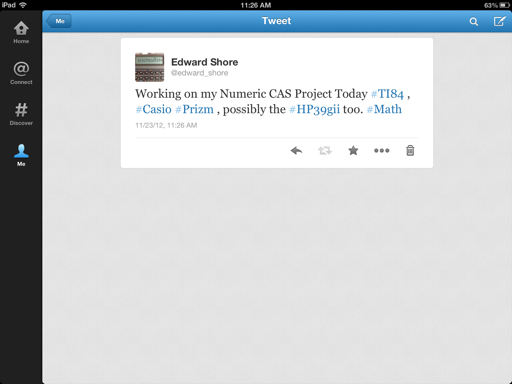## Thursday, November 22, 2012

### Happy Thanksgiving

Wishing everyone a happy and safe Thanksgiving. I am very thankful to all who follow my blog and all who read and make comments. You are truly the best!

May Thanksgiving be a blessed event, memorable, and stress be minimized!

Eddie

## Sunday, November 18, 2012

### Graphing Calculator Programming Languages Comparison: TI 84 Plus vs Casio Prizm

This is a short comparison between the programming languages of the Texas Instruments TI-84+ and the Casio Prizm. For each category, I will list the different syntaxes required.

If you find a program that you like that is programmed on a calculator you don't have, no fear! This guide can be served as a translation guide. For more details, consult the manual or search for detailed tutorials.

Hope this helps,

Eddie

Notes:

The commands for the TI-84+ also covers the TI-84+ Silver Edition, TI-83+ Silver Edition, TI-83+, and most likely the TI-82 and the upcoming TI-84+ Plus C Silver Edition (84+ with a color screen). The TI-84+ family carries a program memory of about 24,000 bytes, 28,000 for the TI-82.

The commands for the Casio Prizm (Model fx-CG 10/20) also apply to the fx-9860g (all versions), fx-9750g, and (most likely) fx-9850g. The Casio family carriers about 60,000-62,000 bytes of program memory, except the 9850g (32,000 bytes).

Keystrokes may vary.

Arguments in each command are in italics.

Here we go:

Numerical Derivative

TI-84+: MATH, 8
nDeriv(f(variable),var,value)

Casio Prizm: OPTN, F4, F2
d/dx(f(X),value)
The variable is always X.

Definite Integral

TI-84+: MATH, 9
fnInt(f(var), var, lower limit, upper limit)

Casio Prizm: OPTN, F4, F4
∫(f(X),lower limit,upper limit)
The variable is always X.

Solve

TI-84+: Catalog or MATH, B in programming mode
solve(expression,variable to be solved for,guess, range*)
expression is set to be equal to 0
range is a two element list {low, high}, and is an optional argument.

Casio Prizm:

There are two commands.

Solve f(X)=0 for X: OPTN, F4, F1
Solve(f(X),guess,low,high)

Solve an equation in any variable: OPTN, F4, F5
SolveN(equation,variable to be solved for,low,high)

Sums

TI-84+: MATH, 0
Σ(f(var),var,start value,end value)

Casio Prizm: OPTN, F4, F6, F3
Σ(f(var),var,start value,end value)

TI-84+:
Input "prompt string", var

Casio Prizm:
"prompt string"? → var

Displaying Results

TI-84+:
Disp var or string
You can add other lines, using commas to separate them.

Pause var
Pause allows the user to scroll the variable's value.

Casio Prizm:
var or string
The ◢ is the right triangle symbol, which acts like a pause command.

If Then Else

TI-84+:
If test expression
Then
do this if test is true
Else
do this if test is false
End

Casio Prizm:
If test expression
Then
do this if test is true
Else
do this if test is false
EndIf

Shortcut: Jump Command
test condition1 command if test is true : skip to here if the test is false

For Loop

TI-84+:
For(counter var,start value,end value,step size*)
commands
End

step size can be positive or negative, and is optional

Casio Prizm:
For start valuevar To end value Step step size*
commands
End

step size can be positive or negative, and is optional

While Loop

TI-84+:
While this test condition is true
do these commands
End

Casio Prizm:
While this test condition is true
do these commands
WhileEnd

Do Until Loop

TI-84+:
Repeat until this condition becomes true
these commands
End

Casio Prizm:
Do
these commands
LpWhile this condition remains false

List and Matrix Element Calls

TI-84+:
Lists:
L#(element number)
#: 1 through 6 or custom name

Matrix:
[[#]](row,column)
#: A through J

Casio Prizm:
Lists:
List #[element number]
#: 1 through 26 or custom name

Matrix:
Mat #[row,column]
#: 1 through 26

Giving the user a menu of options

TI-84+:

Casio Prizm:
Menu "title string", "choice 1", label name...

Decrementing and Incrementing Variables by 1

TI-84+:
IS>(var,target value)
do if var + 1 ≤ target value

DS<(var,target value)
do if var - 1 ≥ target value

Casio Prizm:
ISZ var
do if var + 1 ≠ 0

DSZ var
do if var - 1 ≠ 0

This blog is property of Edward Shore, 2012.

## Sunday, November 11, 2012

### Jacobi Elliptical Functions

This blog entry is possible thanks to @mathematicsprof on Twitter. He posted an article "Jacobi Elliptic Functions from a Dynamic Systems Point of View", written by Ken Meyer Ph.D of the University of Cincinnati, which not only gave me something to read during lunch last Friday but also lead me on how to calculate Jacobi Elliptic Functions. I am so grateful!

By the way, I am on Twitter: @edward_shore.

Jacobi Elliptic Functions - An Introduction

Let k and t be parameters where 0 < k < 1 as the following system of differential equations is to be solved:

dx/dt = y(t) * z(t)
dy/dt = -z(t) * x(t)
dz/dt = -k^2 * x(t) * y(t)

which satisfy the initial conditions x(0) = 0, y(0) = 1, and z(0) = 1.

The Jacobi Elliptical Functions are defined to be the solutions to the above system.

The sine amplitude function is defined as sn(t, k) = x(t).

The cosine amplitude function is defined as cn(t, k) = y(t).

The delta amplitude function is defined as dn(t,k) = z(t).

A very interesting point, which has also managed to trip me up for years, is that there is no explicit closed formula for sn(t,k), cn(t,k), and dn(t,k). Yet mathematicians can find the derivatives and integrals of these functions.

Derivatives of the Jacobi Elliptical Functions

These derivatives, by looking at the system above, are:

d/dt sn(t,k) = cn(t,k) * dn(t,k)
d/dt cn(t,k) = -dn(t,k) * sn(t,k)
d/dt dn(t,k) = -k^2 * sn(t,k) * cn(t,k)

When k=0

By setting k=0 and finding solutions to the system equations becomes:

dz/dt = 0
z(t) = c
Since z(0)=1, conclude z(t)=1.

Then
dx/dt = y(t)
dy/dt = -x(t)
with initial conditions x(0)=0 and y(0)=1.

Since sin(0)=0, cos(0)=1, d/dt sin t = cos t, and d/dt cos t = -sin t, we can conclude that x(t) = sin t and y(t) = cos t. Meyer states that as k approaches 0, sn(t,k) approaches sin(t), cn(t,k) approaches cos(t), and dn(t,k) approaches 1.

A Very Familiar Identity

Similar to the famous identity:

sin²(θ) + cos²(θ) = 1

An identity of Jacobi Elliptical functions is:

sn²(t,k) + cn²(t,k) = 1

Why is this true? Take the derivative with respect to t and we find that:

2 sn(t,k) cn(t,k) dn(t,k) - 2 cn(t,k) dn(t,k) sn(t,k) = 0

How to Calculate

We can use the integral definition to assist us in calculating values for sn, cn, and dn. In this section, let the parameters be u and k, respectively. The integral definition stems from the incomplete elliptical integral: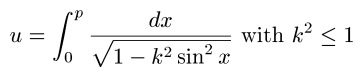where u and k are known and p is the value we are solving for.

The value p is known as the Jacobi Amplitude, am(u,k) for short. Then:

am(u,k) = p

sn(u,k) = sin(am(u,k)) = p

cn(u,k) = cos(am(u,k)) = p

dn(u,k) = √(1 - k² sin² (am(u,k)) = √(1 - k² sin²(p))

Advanced mathematics software or an online calculator, such as this one from Ke!san, are often used to calculate values of Jacobi Elliptical Functions. Fortunately, this task can be done with high-end graphing calculators, using their solve application.

I have been able to obtain accurate answers using the Hewlett Packard HP-50g, Texas Instruments TI-84+, and Casio Prizm fx-CG 10. The later two are relatively fast in finding values. Below I will show the setup for each of the three calculators. I am pretty sure the TI nSpire can handle this too. The following screens show how to obtain am(u,k), the Jacobi Elliptical functions easily follow.

In the solver screen, you can leave X blank (HP-50G only), or just assign any arbitrary value to X, such as 1, since X is the dummy variable in the integration and has no effect on calculations.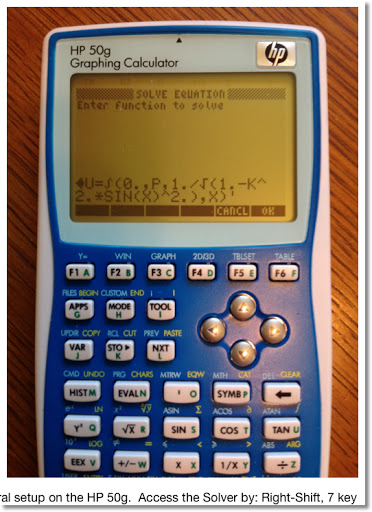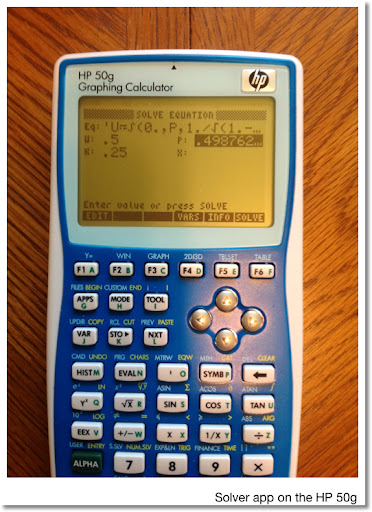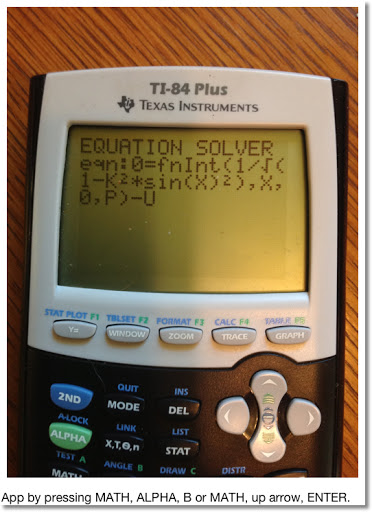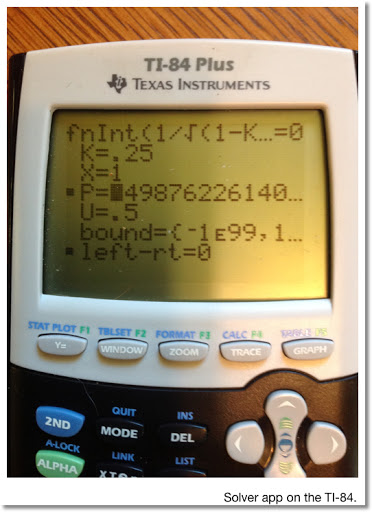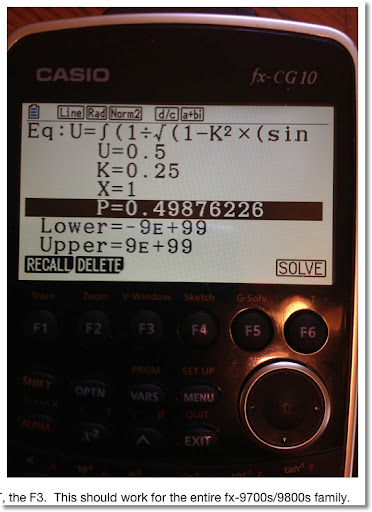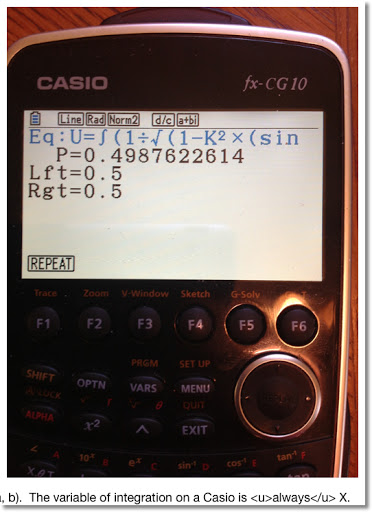Here is a small table of values: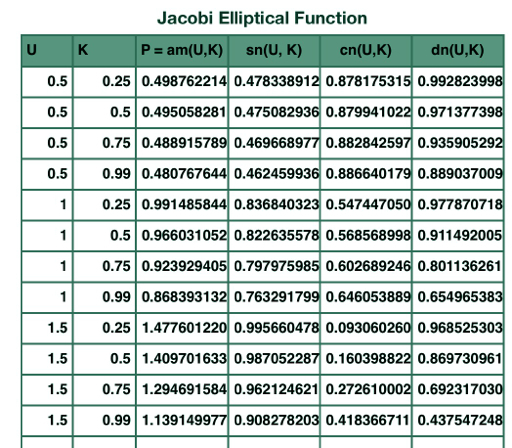Resources

Meyer, Kenneth R. "Jacobi Elliptic Functions from a Dynamic Systems Point of View" The Mathematical Association of America. Monthly 108. October 2001 - retrieved 11/9/2012

Weinstein, Eric "Jacobi Elliptic Functions" - From MathWorld - A Wolfram Web Source, http://mathworld.wolfram.com/JacobiEllipticFunctions.html, retrieved 11/11/2012

Fun as always! Thank you as always. Until next time, Eddie

This blog is property of Edward Shore, 2012.

### The United States Fiscal Cliff: What does it all mean?

Hi everybody. Today I want to talk about tax policy and how the potential tax changes come January 1, 2013 affects taxpayers in the United States.

When the United States Congress goes back into session, one of the hot topics will be the "fiscal cliff". The "fiscal cliff" is a set of income tax credits and tax rates that are set to expire on January 1, 2013; effectively raising the income tax liability for each taxpayer. This blog entry will show how much of a tax increase to expect if no action is taken.

CAUTION AND DISCLAIMER:

1. The information presented today is for general purposes only and is not to be used as income tax advice. If you need advice, please work with a licensed income tax professional.

2. I am only working with federal income tax - be aware that other taxes can increase and decrease.

3. There is no guarantee of the final tax rates for 2013.

4. For today's blog, I will work with single and married filers (married filing jointly). Taxpayers that are head of households, married but filing separately, and other statuses may have different tax rates.

Current United States Tax Law for 2012

These are the current tax laws. When Americans file their income tax early next year, they will calculate tax liability using these rates.

2012 Tax Rates

(income range, tax rate)

Single
\$0-\$8,700; 10% of income
\$8,700-\$35,350; \$870 plus 15% of income excess of \$8,700
\$35,350-\$85,650; \$4,867.50 plus 25% of income excess of \$35,350
\$85,650-\$178,650; \$17,442.50 plus 28% of income excess of \$85,560
\$178,650-\$388,350; \$43,482.50 plus 33% of income excess of \$178,650
\$388,350 and above; \$112,683.50 plus 35% of income excess of \$388,350

Standard Deduction: \$5,950 (phased out as income passes a certain point)

Married Filing Jointly (filing as a couple)
\$0-\$17,400; 10% of income
\$17,400-\$70,700; \$1,740 plus 15% of income excess of \$17,400
\$70,700-\$142,700; \$9,735 plus 25% of income excess of \$70,700
\$142,700-\$217,450; \$27,735 plus 28% of income excess of \$142,700
\$217,450-\$388,350; \$48,665 plus 33% of income excess of \$217,450
\$388,350 and above; \$105,062 plus 35% of income excess of \$388,350

Standard Deduction: \$11,900 (phased out as income passes a certain point)

Personal Exemption: \$3,800 (almost every person gets one)

Let's take an example for a single person in the United States who earned \$50,000. Every taxpayer gets a personal exemption, and assume that this person takes a standard deduction in lieu of itemized deductions. Assuming no other credits apply:

Income: 50,000 - 3,800 - 5,950 = 40,250
Tax Liability for 2012: 4,867.50 + (40,250 - 35,350) * 25% = \$6,092.50

Tax Rates for 2013 if No Action is Taken

If no action is taken, the income tax rates for each bracket will rise, except for the 15% bracket. The 10% bracket disappears entirely. The amounts shown in the table are 2012 amounts to determine the tax brackets - which will most likely be adjusted for inflation. These are estimated tables only, not final.

Tax Increase in 2013?

(income range, tax rage)

Single

\$0-\$35,350; 15% of income
\$35,350-\$85,650; \$5,302.50 plus 28% of income excess of \$35,350
\$85,650-\$178,650; \$19,386.50 plus 31% of income excess of \$85,650
\$178,650-\$388,350; \$48,216.50 plus 36% of income excess of \$178,650
\$388,350 and above; \$123,708.50 plus 39.6% of income excess of \$388,350

Standard Deduction: \$5,950 but will probably increase - final number not released

Married Filing Jointly

\$0-\$70,700; 15% of income
\$70,700-\$142,700; \$10,605 plus 28% of income excess of \$70,700
\$142,700-\$217,450; \$30,765 plus 31% of income excess of \$142,700
\$217,450-\$388,350; \$53,937.50 plus 36% of income excess of \$217,450
\$388,350 and above; \$115,461.50 plus 39.6% of income excess of \$388,350

Standard Deduction: \$9,900 - due to the "marriage penalty"

Personal Exemption: \$3,800 but will most likely increase due to inflation

Let's take the same person, who is Single and makes \$50,000. Assume income level stays level for 2013 and the person takes the standard deduction. Then for 2013:

Income: \$50,000 - \$5,950 - \$3,800 = \$40,250
Estimated Tax Liability for 2013: \$5,302.50 + (\$40,250 - \$35,350) * 28% = \$6,674.50

This represents a potential increase of \$582 in income taxes.

You can use these estimate these tax brackets to estimate the increase in federal income taxes in 2013 - should nothing happen and the Bush Tax Brackets, which were set back in 2001 and 2003, expire.

In addition, the potential tax laws can change in 2013:

* An increase in long term capital gain tax, from 15% to 20%. This tax is for profit made on selling stocks and other long term capital assets held for more than 1 year.
* Social security tax on wages increase from 4.2% to 6.2%. Personally, I see this happening regardless of what happens in Congress over the next two months.
* The exclusion of employer-provided education assistance of \$5,250 can disappear in 2013.
* The American Opportunity Tax Credit revers to the Hope Credit. The credit would be reduced from \$2,500 to \$1,800 and will only be available to students in the first two years of undergraduate education instead of four.
* The Child Credit and Earned Income Tax Credit is set to take a hit for 2013.

Hopefully this will alleviate some fear, or at the very least prepare taxpayers for what could be coming.

What is Congress Discussing

There is talk about extending the Bush tax brackets for at least another year for most taxpayers. Those with the highest income tax brackets (33% and 35%) could see their income tax increase and that what is in debate. Hopefully soon, Americans will know the final income tax structure for 2013

Source:
Discussion by Godfrey Kahn S.C., 5/23/2012 - Retrieved 11/11/2012

Good day everyone. This blog is information purposes only and not to start political debate.

Eddie

## Saturday, November 10, 2012

### Bernoulli Numbers and Polynomials

Today is a good day. I went to the library of alma mater, Cal Poly Pomona for library day. Once a month I try to visit a university library and peruse through with Mathematics Section.I finally got the concept of generating functions. In the many years I studied math, generating functions proved to be an elusive topic for me. Not any more.

What are generating functions?

Generating Functions

Generating functions is a power series. The series is usually does not terminate. The coefficients of the power series can reveal a sequence used in various fields in science.

The Ordinary Generating Function:

G(a_n; x^n) = ∑ a_n * x^n

Sometimes we have an Exponential Generating Function:

E(a_n; x^n) = ∑ a_n * (x^n/n!)

There are other types of generating functions, but I will focus on the basic types described above.

n! represents the factorial of n. In this case, n is a positive integer, and:

n! = n * (n-1) * (n-2) * ... * 3 * 2 * 1

By definition, 0! = 1

To expand the generating function G(a_n; x^n), calculate a Maclaurin Series of G(x). A Maclaurin Series of a function is a Taylor Series about the point x = 0. Hence the Maclaurin Series:

G(x) = G(0) + G'(0) * x/1! + G''(0) * x^2/2! + G'''(0) * x^3/3! + .... + O(x)

where O(x) is the error term G^(n+1)(z) * x^(n+1)/(n+1)! In practice and calculation, O(x) is sometimes "ignored".

An Example: A Basic Generating Function

Expand the generating function, let's go five terms:

G(a_n; x^n) = 1/(1 - x)

G(x) = 1/(1 - x)
Then:
G(0) = 1/(1 - 0) = 1

dG/dx = 1/(x-1)^2; dG/dx(0) = 1

d^2G/dx^2 = -2/(x-1)^3; d^2G/dx^2(0) = 2

d^3G/dx^3 = 6/(x-1)^4; d^3G/dx^3(0) = 6

d^4G/dx^4 = -24/(x-1)^5; d^4G/dx^4(0) = 24

To five terms...

1/(1-x) = 1 + 1 * x/1! + 2 * x^2/2! + 6 *x^3/3! + 24 * x^4/4! + O(x)
= 1 + x + x^2 + x^3 + x^4 + O(x)

The sequence of coefficients are: {1, 1, 1, 1, 1}.

The Bernoulli Numbers

The Bernoulli Numbers can be found by using the Exponential Generating Function:

E(a_n; x) = ∑ x/(e^x - 1)

The Bernoulli Numbers, B_n, are the "coefficients" of the expansion of ∑ x/(e^x - 1). Recall in the Exponential Generating Function, the "coefficients" are of the term x^n/n!.

With E(x) = x/(e^x - 1), the first two derivatives are:

dE/dx = - ((x-1)*e^x + 1)/(e^(2x) - 2*e^x + 1)

d^2E/dx^2 =
((x - 2)*e^(2x) + (x + 2)*e^x) / (e^(3x) - 3*e^(2x) + 3*e^(2x) - 1)

Note that calculating E(0) gives us 0/0. Same for dE/dx(0) and d^2E/dx^2(0). However, if I use a calculate with CAS capabilities such as the Hewlett Packard HP 50g, or mathematical software such as MathStudio (an app for smartphones and iPads), I get something like this (first eight terms):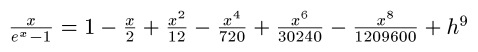Why is that?

Observe that:

lim x/(e^x - 1) as x → 0 = 0/0

Using the L'Hospital's rule, we can take the derivatives of both numerator and denominator,

lim 1/(e^x) as x → 1 = 1

which implies that:

lim x/(e^x - 1) as x → 0 = 1

You can generate terms by taking the limit as x → 0 for each term.

This how we end up with the series. Now to extract the "coefficients", observe that:

12 = 6 * 2!
(no term contains x^3/3!)
720 = 30 * 4!
(no term contains x^5/5!)
30240 = 42 * 6!
(no term contains x^7/7!)
1,209,600 = 30 * 8!

Our sequence for this generating function (for nine terms) is:

{1, -1/2, 1/6, 0, -1/30, 0, 1/42, 0, -1/30}

These numbers are the Bernoulli numbers. In fact, definition by generating function is:

x/(e^x - 1) = ∑ B_n * (x^n/n!)

Bernoulli Numbers

B_0 = 1
B_1 = -1
B_2 = 1/6
B_3 = 0
B_4 = -1/30
B_6 = 1/42
B_8 = -1/30
B_10 = 5/66
B_12 = -691/2730
B_14 = 7/6

B_n = 0 where n is odd and n > 2

Bernoulli Polynomials

Bernoulli Polynomials can be generated by the following formula:

β_n(x) = ∑((B_k * n!/(k!*(n-k)!) * x^k, from k = 0 to n)

where B_k is the kth Bernoulli number.

Source:
Krylov, Vladimir Ivanoch, translated by H. Stroud Approximate Calculations of Integrals McMillian Company: New York, 1962

Until next time, be safe everyone! Eddie

This blog is property of Edward Shore, 2012.

## Friday, November 9, 2012

### The Sine Wave - A Portrait

Good Friday!

One of my favorite mathematical functions is the sine function. Not only the sine wave helps describe a lot of physical phenomena, but I also see it like how life goes, with its ups and downs.

So here are few graphs (portraits) of the sine wave.

Settings:
Calculator: TI-84+
Window: X range: -π/2 to 5π/2, Y range: -2.05 to 2.05
(Except the last one, X range: 0 to 5π/2)

Eddie

This blog is property of Edward Shore, 2012.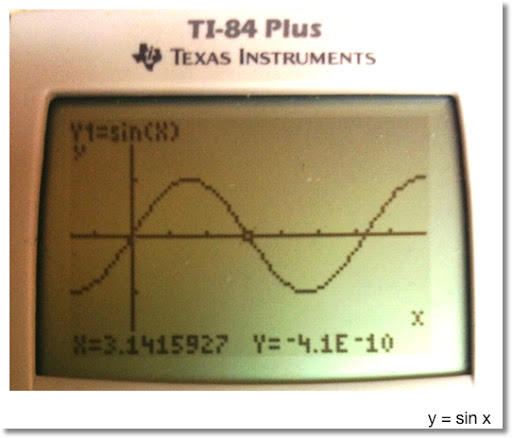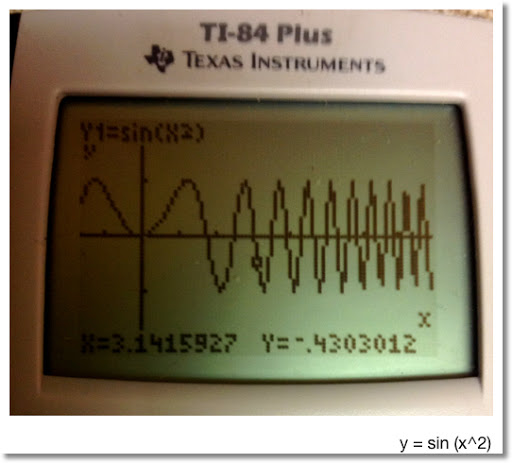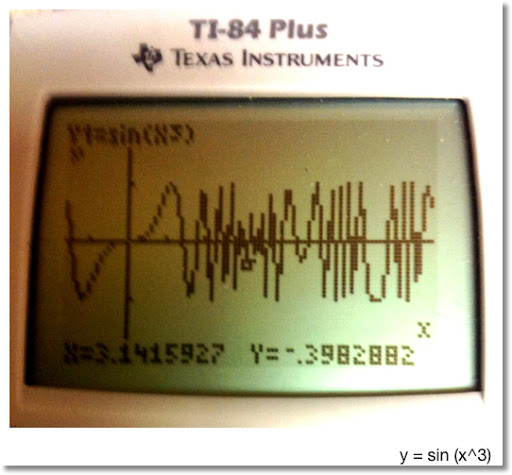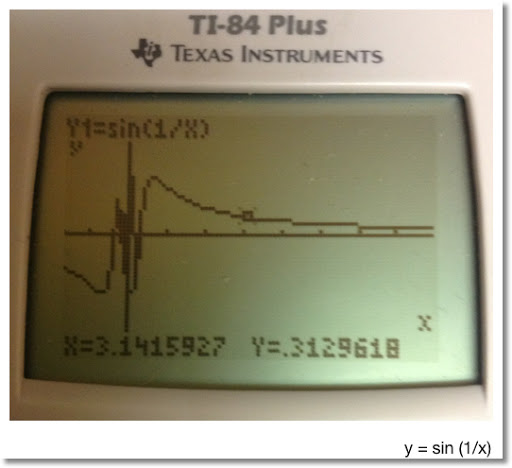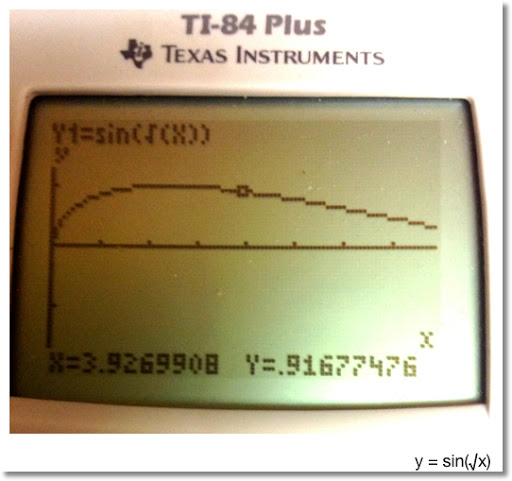## Sunday, October 28, 2012

### Review of FreeCalcFxc

Review: FreeCalcFxC

Platforms: iPhone and iPod Touch, will run on an iPad

Versions Available:
Free Version
\$0.99 Scientific Version
\$0.99 Financial Version

The only difference between the Free Version and the Scientific Version is that the Free Version is ad-supported.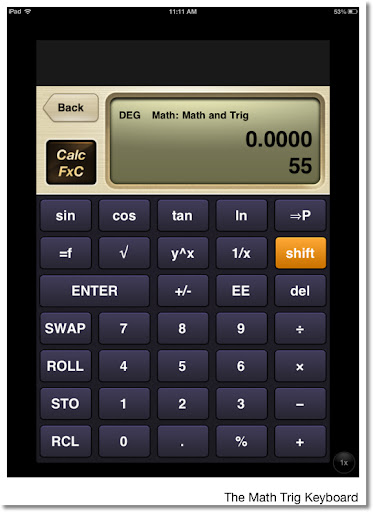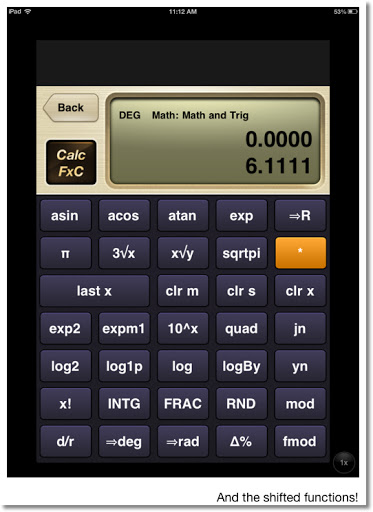Keyboard

The keyboard is a simple layout of 34 keys. The keys are labeled with one function. To access the shift function, press the gold shift key. The names of the shifted function replace the primary functions. Kudos for simplicity, however, my personal preference is to see both the primary and shift functions at the same time.

RPN and Algebraic Mode

The default mode is RPN (Reverse Polish Notation), but you can always enter an algebraic formula by pressing the "=f" key. The "=f" becomes the "=" to terminate entry of the formula.

Many, many, many calculators are available

Accessing the Focused Calculator list gives many calculators such as Math and Trig (the main calculator), Time Value of Money, Bonds, Statistics, Probability and Conversion calculators. For me the Conversion calculators leave a little to be desired, since there are no direct conversion keys attached to the default keyboard (luckily, that can be remedied!).

In addition to the Focused Calculators, CalcFxC gives formula template calculates. Among these formula calculators you have Percent Change, Distribution Functions, and the Quadratic and Cubic Equations.

The keyboard gives a good response when the "keys" are pressed. The screen is a two line (adjustable) screen which displays the y-stack and x-stack. The stack is four levels.

Help can be accessed by pressing and holding a key.

Real Numbers and Limits

The calculator operates on real numbers only. So, entering √-1 will return an error. As a consequence, the polynomial solvers return only real roots. The numbers range in the order of -10^-308 to 10^308.

There are no fractions or exact values of trig functions.

Math and Trig Keyboard

sqrtpi: takes the square root of the number then multiplies it by π
exmp1: e^x - 1, known on Hewlett Packard Calculators as the EXM1 function
log1p: ln(x + 1), known on Hewlett Packard Calculators as the LNP1 function
jn: Bessel Function of the First Kind, with x on the y-stack and the order n on the x-stack. jn also returns the Bessel Function of the Second Kind.
quad: Takes three arguments from the stack (a, b, c of ax^2+bx+c) and returns the real roots.

If you want to access the hyperbolic functions, you will need to call the Math and Hyperbolic calculator.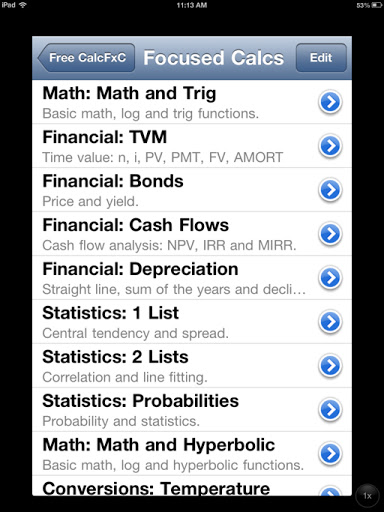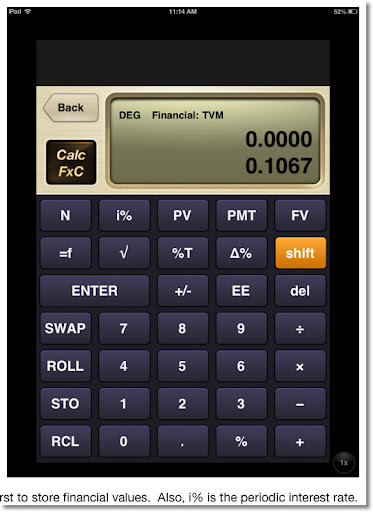Memory

The calculator has 27 memories: memories a through z, and a special register ra. Storage and recall arithmetic can be performed on register ra - no idea why (except for maybe programming limitations) CalcFxC decided to restrict this feature to one register.

Programmability in the Form of Customizable Calculators

CalcFxC does not offer "traditional" macro or program capability. Instead, CalcFxC offers the ability to edit and create custom keyboards. You can redefine the key's name and help screen, along with it's formula. You have access to all of the functions. To use the stack arguments, use rgx(), rgy(), rgz(), and rgt() for the x, y, z, and t stacks respectively. This is a fun feature for those who has ever wanted to design their own calculators, and I am one of them!

To emulate the stack operations properly, you will need to define the formulas for each stack.

For example, if I designate the comb function as the Combination function, I would use the following formulas:
x Formula: combin(rgy(),rgx())
y Formula: rgz()
z Formula: rgt()
t Formula: rgt()
Last x Formula: rgx()

Functions can be copied and pasted. You will need some experience with RPN calculators to take advantage of this feature.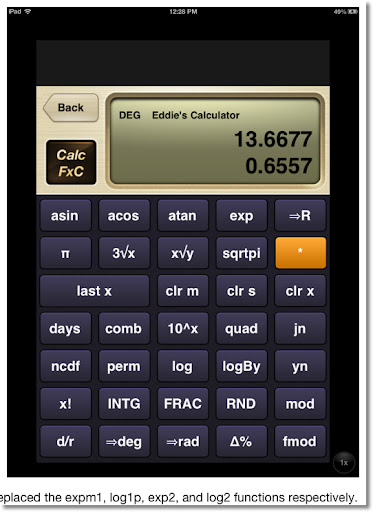Final Word

This app is enjoyable to use. I am probably going to spend an afternoon or evening making a custom calculator. RPN fans, this is a good calculator app to get.

Since I am not a fan of ads, I will pay the \$0.99 to get the non-ad version.

4.5 of 5 stars.

Eddie

This blog is property of Edward Shore. 2012.

## Wednesday, October 24, 2012

### Permutations: When Objects Repeat

Monday's blog entry (10/22/2012), Factorials and Arrangements of Unique Objects dealt with permutations of arranging a group of objects where all the objects are unique. Today's blog entry looks at three situations where objects can be repeated.

All the Choices are Available all the Time

This is where you make permutations in which all the objects are available for each slot. For example, let's take a five digit zip code. There are five slots and for each slot the 10 digits are available: 0, 1, 2, 3, 4, 5, 6, 7, 8, and 9.

For the first slot there are 10 choices. For each of the 10 choices, the second slot presents another 10 choices. For each of those 10 choices, the third slot presents another 10 choices. And so on.

The number of arrangements is: 10 × 10 × 10 × 10 × 10 = 100,000. There are 100,000 five-digit zip codes possible, 00000 to 99999.

Remember when calculating permutations, order matters. For our example, 11110, 11101, 11011, 10111, and 01111 are five different arrangements. The general rule is presented below.

Permutations where all the choices are available all the time = n^k

where
n = number of objects
k = number of slots

Objects Can Repeat, but with Some Restrictions

This is similar to the first situation in the way of calculating the number of permutations (arrangements).

Let's say we are on a very famous game show. One of it's mini games is played for a car valued anywhere from \$20,000 to \$59,999. We want to know how many possible prices for this game are possible.

Looking at the range from \$20,000 to \$59,999: we can see the choices for each of the five digits. The first digit must be a 2, 3, 4, or 5. The other four digits can be anything 0 through 9 (10 choices each).

The number of prices possible are 4 × 10 × 10 × 10 × 10 = 40,000.

So using a random guess, the contestant has a 1 in 40,000 choice in getting the price exactly right.

Another mini-game offers cars from \$11,111 to \$36,666 where the first digit is given to the contestant (1, 2, or 3) and the contestant tries to roll the other four digits using a single die. The die contains the numbers 1, 2, 3, 4, 5, and 6. Our task to find out how many prices are possible.

There are 3 choices for the first digit, and 6 choices for the other four digits.

The number of prices possible are 3 × 6 × 6 × 6 × 6 = 3,888.

This mini-game can be played up to 3,888 times before a price repeats.

Permutations where:
1) Different slots can have restrictions
2) Each choice is independent

P = (number of choices for slot 1) × (number of choices for slot 2) × ... × (number of choices for slot k)

Rearranging a Group of Objects where some of the Objects Repeat

In this situation we are finding the number of permutations of a group of objects, except some of the objects repeat.

Let's try to find the number of ways to arrange the letters in the word PHYSICS, removing any restriction that the arrangement has to make a sensible word (HYSSICP would count as arrangement).

In the word PHYSICS, there is 1 "P", 1 "H", 1 "Y", 2 "S"s, 1 "I", and 1 "C", for a total of 7 letters. PHYSICS counts as one permutation, regardless which "S" is used in each slot. We still have 7 letters, which can be arranged 7! = 5,040 ways, but have to account for the 2 "S"s.

The true number of ways to arrange the letters in the word PHYSICS is 2,520 ways.

7! / 2! = 5,040/2 = 2,520

Coincidentally, the calculation is really 7! / (1! × 1! × 1! × 2! × 1! × 1!). However, 1! = 1. Hence, 1! × 1! × 1! × 2! × 1! × 1! = 2!.

Let's take another example. Find the number of ways to arrange the letters in the "word" AAABB. In this example, there are 3 "A"s and 2 "B"s for a total of 5 letters.

If all the letters were unique, the number of ways is 5!. But we have to account for the repeats. Divide by 3! for the "A"s and 2! for the "B"s. The result is:

5! / (3! × 2!) = 120 / (6 × 2) = 10

There are only 10 ways to arrange the letters of the "word" AAABB. The ten are:

AAABB
AABAB
AABBA
ABABA
ABBAA

BABAA
BBAAA
ABBAA
AABBA
BAAAB

Finding the Number of Arrangements of Objects Where Some Objects Repeat

n! / ( (k_1)! × (k_2)! × ... × (k_m)! )

where:
n = the total number of objects, including repeated objects
m = the number of unique objects
k_1 = number of "k_1" objects
k_2 = number of "k_2" objects
and so on until...
k_m = number of "k_m" objects

The expression above is known as a multinomial coefficient.

I hope this day is well for each of you. Thank you for your comments and suggestions. Take care,

Eddie

Source:
Marcus, Daniel A. "Combinatorics: A Problem Orientated Approach" Mathematical Association of America, Washington DC. 1998.

This blog is property of Edward Shore, 2012.

## Tuesday, October 23, 2012

### Factorials and Arrangements of Unique Objects

Last weekend my dad asked me about my blog, and the last entry was about factorials of large numbers. Clicking on this link will take you there. On our way to classic car auto shop in Orange, CA; my dad and I talked about the factorials as I tried to come up with a way of finding applications using factorials. Last weekend became the inspiration for my blog entry. Love you, Dad.

Note: This blog entry will cover factorials of non-zero integers, that is n = 0, 1, 2, 3...

Factorials

The factorial of a non-negative integer, written with an exclamation mark after the number, is defined as:

n! = n × (n - 1) × (n - 2) × (n - 3) × ... × 3 × 2 × 1

Start by multiplying n by n less 1, then multiplying the product by n less 2, and repeat until you get to 1.

Examples
5! = 5 × 4 × 3 × 2 × 1 = 120
8! = 8 × 7 × 6 × 5 × 4 × 3 × 2 × 1 = 40,320

By definition, 0! = 1. Dr. James Tanton, Ph. D (www.jamestaton.com) gives an explanation why mathematicians choose to in video: Link to Video

Simply put, 0! = 1 is just defined this way.

Arrangements

In this section, we consider three common arrangement problems. The task is to find the number of possible permutations a group of objects can be arranged. A permutation is an arrangement where the order of which the objects are placed is important.

In this section, we are going to arrange all the objects.

Arrangement of Unique Objects

Consider a bookshelf that has room for five books. For simplicity, let's call the books A, B, C, D, and E (and not "Combinatorics", "The Irrationals", "Euclid's Number", "Programming", and "Making Soup for Dummies" like I originally planned.)

The for the first slot there are five choices. The second slot provides four choices. Whatever is available for the second slot depends on what book was put in the first slot. For example, if I choose to put book A in the first slot, the books B, C, D, and E are available for the second slot. Instead, if I choose to put book C in the first slot, then A, B, D, and E are available.

For each choice I make on the first slot, I get four choices for the second. Considering the first two slots alone, this gives me a total of 5 × 4 = 20 arrangements.

Continuing in this way, there are three choices for the third slot, two choices for the fourth slot, and whatever is left over gets the fifth slot.

So, the total number of arrangements of five books is:

5 × 4 × 3 × 2 × 1 = 5! = 120

Yes, 120 different arrangements. Since order is important, the arrangement is considered a permutation.

In general, working with n objects and n slots:
There are n objects for the first slot,
there are n - 1 objects for the second slot,
there are n - 2 objects for the third slot,
and so on,
until we reach 1 slot left for the last object.

Hence, the number of arrangements are:

n × (n - 1) × (n - 2) × ... × 1 = n!

Number of Permutations of n Unique Objects = n!

Arrangement of Unique Slots, Limited Spaces Available

Let's go back to our problem of arranging five books (A, B, C, D, and E) but this time, we only have three slots available.

For the first slot, I have 5 books available to choose from. Depending on what I choose, I will have 4 books for the second slot. Each of those 4 books present a choice of the 3 books for the last slot. Whatever is left either goes somewhere else in the house or gets donated.

The number of arrangements that are available to me has decreased due to the fact I only have three slots available. Hence, 5 × 4 × 3 = 60.

Observe if we multiply 5 × 4 × 3 by (2 × 1)/(2 × 1) we get:

5 × 4 × 3
= (5 × 4 × 3 × 2 × 1) / (2 × 1)
= 5! / (2 × 1)
= 5! / (5 - 3)!

Basically we are taking all of the ways 5 books can be arranged, and then dividing that number by all the ways the 2 books that are not going to be used can be arranged.

Doing the above in the general case is how we arrive at the formula for permutations:

nPk = n! / (n - k)!

where we have n objects and k slots to fill.

Permutations: nPk = n! / (n - k)! where n ≥ k. Order is important.

Until next time, have a great day!

Eddie

This blog is property of Edward Shore, 2012.

## Saturday, October 20, 2012

### Factorial of Large Numbers

Hi everyone! I am blogging from Coffee Klatch in San Dimas, CA! I was overdue for a latte. Their pumpkin brownies are to die for.

Factorial of Large Numbers

Calculators have an upper limit when working with numbers. Typically, the scientific calculators allow numbers up to 9.999999999 × 10^99. Anything over 10^100 is considered an overflow. Some of the higher graphing and CAS calculators allow for higher limits.

This means if you need to calculate anything over 69!, most calculators will give an "Overflow" error message.

Factorial:

The factorial of an integer n is the product:

n! = n × (n - 1) × (n - 2) × ... × 3 × 2 × 1

We can remedy this by using the laws of logarithms to calculate factorials of higher integers. Common logarithms (base 10) are used.

n! = n × (n - 1) × (n - 2) × ... × 2 × 1
log (n!) = log [ n × (n - 1) × (n - 2) × ... × 2 × 1 ]
log (n!) = log (n) + log (n - 1) + log (n - 2) + ... + log (2) + log (1)

log (n!) = ∑( log(k) from k = 2 to n)

Let T be this sum.

T = ∑(log (k) from k = 2 to n)

Note the following laws are used:

log (a × b) = log a + log b
log (1) = 0

If we take the anti-common logarithm of both sides, we get:

n! = 10^(∑( log(k) from k = 2 to n)
n! = 10^(T)
n! = 10^(frac(T) + int(T))
n! = 10^(frac(T)) × 10^(int(T))

Where:

(1) int(T) is the integer part of T. To find it, take the integer and ignore everything after the decimal point.

(2) frac(T) is the fractional part of T. To find this, take everything after the decimal point and ignore the integer part.

(3) int(T) + frac(T) = T

Example:
int(14.125) = 14
frac(14.125) = 0.125

A lot of programming and graphing scientific calculators have the int and frac functions. On most Hewlett Packard programming and scientific calculators, they are named IP and FP, respectively.

Example 1: 8!

Calculate 8! using the logarithm method.

T = ∑( log(k) for k = 2 to 8) = log 2 + log 3 + log 4 + log 5 + log 6 + log 7 + log 8 ≈ 4.605520523

In this case, int(T) = 4 and frac(T) = 0.605520523.

Then 8! ≈ 10^(0.65520523) × 10^4 ≈ 4.031999996 × 10^4

Because the accuracy of calculators and computer programs, I recommend that you round 10^(frac(T)) to a set amount of decimal places. I find that 5 decimal places works well. Then:

8! ≈ 4.03200 × 10^4

In fact, 8! = 40,320

Example 2: 111!

1. Calculate the sum ∑( log(k) from k=2 to 111). We find the answer to be 180.2462406.

2. Split the integer and fractional portions. Take the anti-common logarithm of the fractional portion. Hence, 10^0.2462406 ≈ 1.762952551. I round this answer to five decimal places to get 1.76295.

3. Answer: 111! ≈ 1.76295 × 10^180

In fact, using an HP 50g, 111! =
1,762,952,255,109,024,466,387,216,104,710,707,578,876,140,953,602,656,551,604,157,406,334,734,695,508,724,831,643,655,557,459,846,231,577,319,604,766,283,797,891,314,584,749,719,987,162,332,009,625,414,533,120,000,000,000,000,000,000,000,000

And yes, typing out this long number is not as easy it sounds.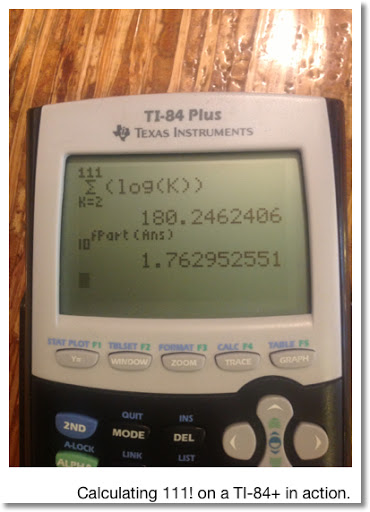Programming

Now we come to the programming portion of calculating n! using the logarithmic method.

This program was done on a HP 39gii, but can easily adapted on most graphing/programming calculators.

Program LFACT

EXPORT LFACT(N)
BEGIN
LOCAL T,M;
Σ(LOG(K)),K,2,N)→T;
ROUND(10^FRAC(T),5)→M;
MSGBOX(M + "10^" + INT(T));
RETURN {M, INT(T)};
END;

Here is a program in BASIC language. I used the App techBASIC from ByteWorks.
Everything after explanation points signify comments.

! Approximate Factorial (5 decimal mantissa plus exponent)
! Eddie Shore
! 10/20/2012

! To improve accuracy, set all variables as DOUBLE
01 DIM N as DOUBLE
02 DIM T as DOUBLE
03 DIM F as DOUBLE
04 DIM M as DOUBLE
05 DIM K as DOUBLE

10 PRINT "Factorial Program"
11 INPUT "N = "; N
12 T = 0

! Sum Loop
14 FOR K = 2 TO N
16 T = T + LOG10(K)
18 NEXT K

! Separation of T into its fractional and integer parts, we know T>0
20 M = INT(T)
22 F = T - M

! Round Fraction to five places - we have no ROUND function to work with
! 10^x when x<1 has a value less than 10
! Use string functions to round the answer to 5 decimal places (7 characters: the integer, decimal point, and five decimal places)
30 F = 10^F
32 F = VAL(LEFT(STR(F),7))

! Final results
40 PRINT "N! = "; F ; " x 10^"; M

Test Data:
8! = 4.0320 × 10^4
66! = 5.4434 × 10^92
122! = 9.87504 × 10^202
425! = 1.59775 × 10^934

Thank you as always, I appreciate the comments and compliments. Hope you enjoy the weekend.

Until next time,

Eddie

This blog is property of Edward Shore, 2012.

### HP 42S/DM42: Height of a Fire, Time to Clear a Corridor

HP 42S/DM42:  Height of a Fire, Time to Clear a Corridor HP 42S/DM42/Free42 Program:  FIREHGT The program FHGT approximates the height of a...